Home Up Questions?

Chapter 5. Complexity and Decidability

In Chapter 4 we presented a number of problems which have been defined for Petri nets. These problems concern various properties of Petri net structure and behavior which, under appropriate circumstances, would be of interest to users of Petri nets.

Two solution techniques were also presented: the reachability tree and matrix equation approaches. These two techniques allow properties of safeness, boundedness, conservation, and coverability to be determined for Petri nets. Also, a necessary condition for reachability was established. However, these analysis techniques are not sufficient to solve several other problems, especially liveness, reachability, and equivalence. In this chapter we explore these problems, either to find solutions to them or at least to learn more about the properties of Petri nets.

5.1. Reducibility Between Analysis Problems

A fundamental concept which we use is reducibility [Karp 1972]. Solving a problem involves reducing it to another problem which we already know how to solve. For example, in the previous chapter, the problem of determining if a Petri net is conservative was reduced to solving a set of simultaneous linear equations. The problem of solving sets of simultaneous linear equations has in turn been reduced to a defined sequence of arithmetic operations (addition, subtraction, multiplication, division, and comparisons). Thus, since the simpler arithmetic operations can be computed, conservation can be determined.

Another example concerns the equality problem and subset problem for reachability sets.

DEFINITION 5.1 Equality Problem
Given two marked Petri nets C1 = (P1, T1, I1, O1 ) with marking μ1 and C2 = (P2, T2, I2, O2 ) with marking μ2, is R ( C1, μ1 ) = R ( C2, μ2 ) ?
DEFINITION 5.2 Subset Problem
Given two marked Petri nets C1 = (P1, T1, I1, O1 ) with marking μ1 and C2 = (P2, T2, I2, O2 ) with marking μ2, is R ( C1, μ1 ) ⊆ R ( C2, μ2 ) ?
These two problems can be very important if Petri nets are to be “optimized” or if the nets of two systems are to be compared. However, notice that if a solution to the subset problem can be found, the equality problem is also solved. If we wish to determine if R ( C1, μ1 ) = R ( C2, μ2 ), we can first use the subset problem algorithm to determine if R ( C1, μ1 ) ⊆ R ( C2, μ2 ), and then use the same algorithm to determine if R ( C2, μ2 ) ⊆ R ( C1, μ1 ) . R ( C1, μ1 ) = R ( C1, μ2 ) if and only if R ( C1, μ1 ) ⊆ R ( C2, μ2 ) and R ( C2, μ2 ) ⊆ R ( C1, μ1 ) . Thus, we can reduce the equality problem to the subset problem.

Two other considerations are of importance when considering analysis problems and reducibility. First, in trying to find a solution, we must consider the possibility that a problem has no solution technique; it is undecidable. Second, if a solution technique exists, we need to consider its cost: How much time and memory space are needed? For Petri nets to gain widespread general use, analysis problems must be solvable and by algorithms which are not excessively expensive in computer time or space.

Reducibility plays a role in both of these problems. Reducibility between problems is commonly used to show that a problem is decidable or undecidable. Our approach to decidability theory [Davis 1958; Minsky 1967] is based mainly on the work of Turing and on his model of computations, the Turing machine. The importance of the Turing machine is that it is a reasonable representation of a limited computing machine and that it can be shown that no algorithm exists which can solve certain Turing machine problems, especially the halting problem. From this basis, a collection of undecidable problems has been found. The importance of this theory is that it is not possible to produce a computer program which solves these problems. Thus, for practical analysis, these undecidable problems must be avoided, or the analysis questions will be unanswerable.

(An important distinction here is that undecidable problems produce questions which are not simply unanswered but unanswerable. Questions can be unanswered but still answerable; this merely means that no one has yet found an answer but that the answer does exist. A famous example is Fermat's last theorem: Does the equation xn + yn = zn have solutions for n > 2 and nontrivial integer x, y, and z? This question has not been answered, but it is answerable. The answer is either yes or no. One way to answer the question is to produce numbers x, y, z, and n which satisfy the theorem. The other way would be to prove (logically deduce) that no such x, y, z, and n can exist. No one has yet done so.

However, assume that the problem were undecidable. Then it is not possible to decide whether x, y, z, and n exist which solve the equation. This means we could not logically deduce their nonexistence from the axioms of mathematics and that we cannot produce x, y, z, and n which solve the equation. But if we cannot produce x, y, z, and n, then they must not exist. If they did exist, we could set a computer to searching for them, and, eventually, it would find them. But if x, y, z, and n do not exist, then the answer to the question is no, and we have decided it. This contradicts our assumption that the question is undecidable, so the question is decidable.)

Now assume that a problem A is reducible to a problem B: An instance of problem A can be transformed into an instance of problem B. If problem B is decidable, then problem A is decidable, and the algorithm for problem B can be used to solve problem A. An instance of problem A can be solved by transforming it to an instance of problem B and applying the algorithm for problem B to determine the solution. Thus, if problem A is reducible to problem B and problem B is decidable, then problem A is decidable.

The contrapositive is also true: If problem A is reducible to problem B and problem A is undecidable, then problem B is undecidable; for if problem B were decidable, the above procedure is a decision technique for problem A, contradicting its undecidability. These two facts are central to most decidability techniques. To show that a problem is decidable, reduce it to a problem which is known to be decidable; to show that a problem is undecidable, reduce a problem which is known to be undecidable to it.

We shall make good use of this approach to reduce the amount of work we must do. For example, since the equality problem for reachability sets is reducible to the subset problem, we want to develop either (1) a solution procedure for the subset problem or (2) a proof that the equality problem is undecidable. If we can show (1), we have a solution technique for both problems; if we show (2), we know both problems are undecidable.

In some cases, we may be able to do even better. Two problems are equivalent if they are mutually reducible. That is, problem A is equivalent to problem B if problem A is reducible to problem B, and problem B is reducible to problem A. In this case, either both problems are decidable or both are undecidable, and we can work on either one. (Notice that this is not true in general. For example, if we were to show that the subset problem for reachability sets is undecidable, this would tell us nothing about the decidability or undecidability of the equality problem.)

The second consideration for investigating analysis problems is that if a solution technique exists it must be reasonably efficient. This requires that the amount of time and memory space needed by an algorithm to solve an instance of the problem not be excessive. The study of the cost of executing an algorithm is a part of complexity theory. Complexity theory deals with the amount of time and space needed to solve a problem. Obviously the amount of time and space will not be constant but will vary with the size of the problem to be solved. For Petri nets, time and space requirements would probably be a function of the number of places and transitions. Other factors which might influence things would be the number of tokens in the initial marking or the number of inputs and outputs for each transition and place (the number of arcs in the graph).

The time and space needed will vary with the particular instance of the problem to be solved. Therefore, complexity results may be in the form of a best case (lower bound) or worst case (upper bound) for an algorithm. Since it is not known in advance whether an instance will be a best case or worst case, the worst case is generally assumed, and the complexity of an algorithm is the worst case time or space requirements, as a function of the size of the input.

Complexity analysis is mainly concerned with the underlying problem complexity, and not concerned with a specific detailed implementation of any particular algorithm. Thus, complexity theory ignores constant factors. Complexity for a problem of size n is determined to be of order n2 or en or n log n allowing for smaller terms and constant factors. In particular two general classes of algorithms are important: those with polynomial complexity ( n, n2, n log n, n8, and so on) and those with nonpolynomial complexity (especially exponential, 2n, and factorial, n ! ).

Complexity analysis is generally applied to specific algorithms but can also be applied to general problems. In this case, a lower bound on the complexity of all algorithms to solve a problem is determined. This provides an algorithm-independent complexity result. It also can be useful in showing that a particular algorithm is optimal (within a constant) and when further work may produce a significantly better algorithm to solve a problem. For example, it is well-known that sorting n numbers is of complexity n log n. Thus algorithms with n log n complexity cannot be significantly improved on (in the asymptotic worst case).

Reducibility can be useful in determining complexity. If a problem A can be reduced to a problem B and B has a complexity fB ( n ), then the complexity of A is at most the complexity of B plus the cost of the transformation from A to B (keeping in mind that the size of the problem may also change in the transformation). The complexity of the transformations is generally constant or linear and so is often ignored. Thus, reducing problem A to problem B gives either an upper bound for the complexity of A (if the complexity of B is known) or a lower bound for the complexity of B (if the complexity of A is known). Again by using as an example the equality and subset problems, the amount of work needed to solve the equality problem is no greater than twice the amount of work for the subset problem. Since this is a constant factor, the complexity of the subset problem should be the same as the complexity of the equality problem.

These two properties of Petri net analysis properties -- decidability and complexity -- are of major concern for the use of Petri nets. In this chapter we present some results which have been obtained. One of the techniques used is to reduce one Petri net problem to another.

5.2. Reachability Problems

The reachability problem is one of the most important problems for Petri net analysis. It is also open to a large amount of variation in definition. The following four reachability problems for a Petri net C = (P, T, I, O) with initial marking μ have been posed.

DEFINITION 5.3 The Reachability Problem
Given μ′, is μ′ ∈ R ( C, μ ) ?
DEFINITION 5.4 The Submarking Reachability Problem
For a subset P′ ⊆ P and a marking μ′, does there exist μ′′ ∈ R ( C, μ ) such that μ′′ ( pi ) = μ′ ( pi ) for all piP′ ?
DEFINITION 5.5 The Zero-Reachability Problem
Is μ′ ∈ R ( C, μ ) with μ′ ( pi ) = 0 for all piP? [Is 0 ∈ R ( C, μ ) ?]
DEFINITION 5.6 The Single-Place Zero-Reachability Problem
For a given place piP, does there exist μ′ ∈ R ( C, μ ) with μ′ ( pi ) = 0 ?
The submarking reachability problem restricts the reachability problem to considering only a subset of places, not caring about the markings of other places. The zero-reachability problem asks if the specific marking with zero tokens in all places is reachable. The single-place zero-reachability problem asks if it is possible to empty all the tokens out of a particular place.

Although these four problems are all different, they are all equivalent. Certain relationships are immediately obvious. The zero-reachability problem is reducible to the reachability problem; we simply set μ′ = 0 for the reachability problem. Similarly the reachability problem is reducible to the submarking reachability problem, by setting the subset P′ = P. The single-place zero-reachability problem is reducible to the submarking reachability problem by setting P′ = { pi } and μ′ = 0 . More difficult to show is that the submarking reachability problem is reducible to the zero-reachability problem and that the zero-reachability problem is reducible to the single-place zero-reachability problem. This entire set of relationships is shown in Figure 5.1.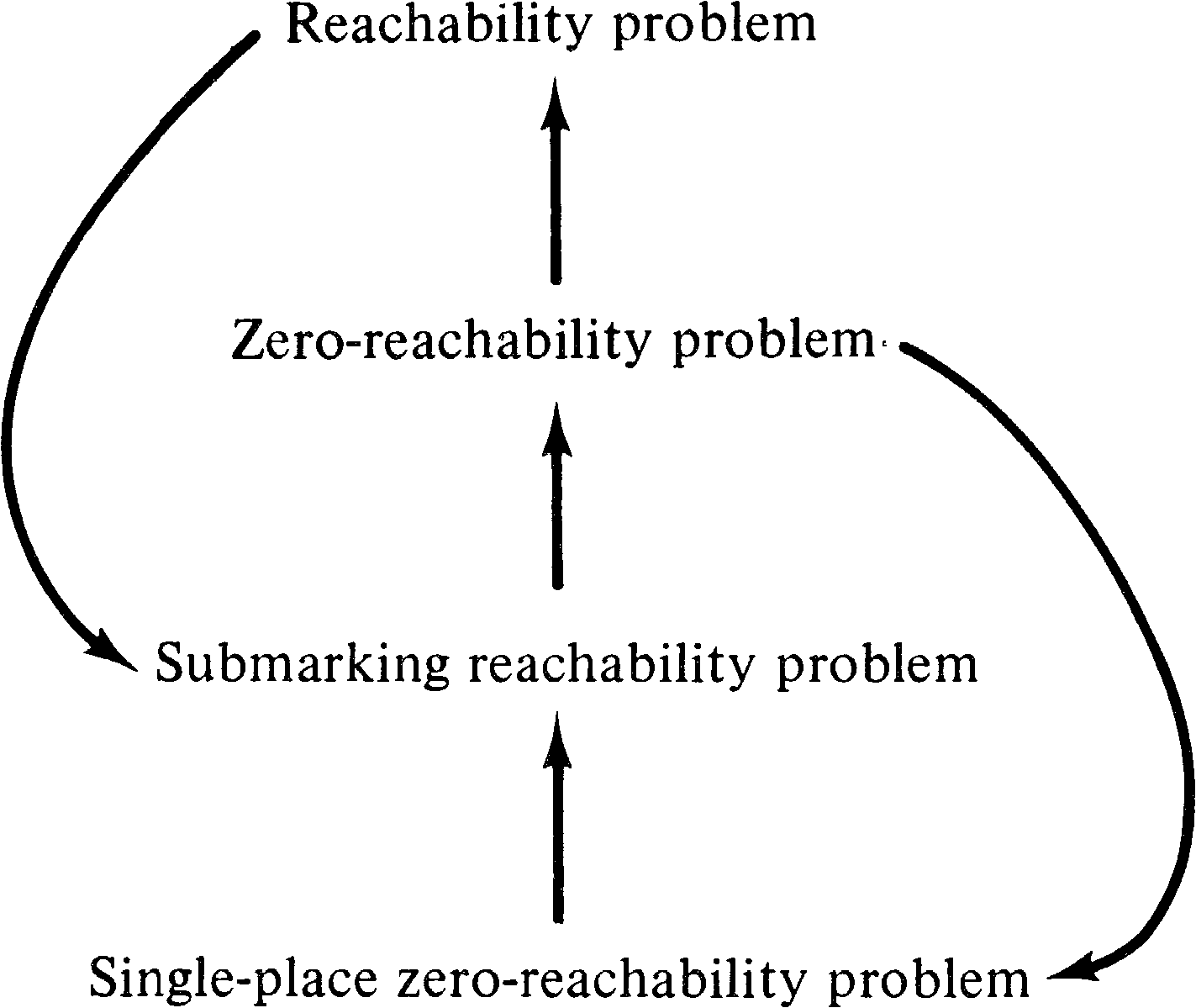Figure 5.1. Reducibility among reachability problems. An arc from one problem to another indicates that the first is reducible to the second.

First, we show that the submarking reachability problem is reducible to the zero-reachability problem. Assume we are given a Petri net C1 = (P1, T1, I1, O1 ) with initial marking μ1, a subset of places P′ ⊆ P1, and a marking μ′ . We want to know if there exists μ′′ ∈ R ( C1, μ1 ) with μ′ ( pi ) = μ′′ ( pi ) for all piP′ . Our approach is to create a new Petri net C2 = (P2, T2, I2, O2 ) with initial marking μ2 such that there exists μ′′ ∈ R ( C1, μ1 ) with μ′ ( pi ) = μ′′ ( pi ) for all piP′ if and only if 0 ∈ R ( C2, μ2 ) .

The construction of C2 from C1 is quite straightforward. We start with C2 the same as C1. To allow any place pi not in P′ to become empty we add a transition ti′ with input { pi } and null output. This transition can fire whenever there is a token in pi to drain off any tokens which may reside here. This allows us to ignore these places, being sure that they can always reach a zero marking.

For places pi in P′, we must assure that exactly μ′ ( pi ) tokens are in pi. To assure this we create a new place pi′ for each piP′ with an initial marking of μ′ ( pi ) tokens and a transition ti′ with input { pi, pi′ } and null output. If there are exactly μ′ ( pi ) tokens in pi, then this transition can fire exactly μ′ ( pi ) times, reducing the markings of pi and pi′ to zero. If the number of tokens in pi is not μ′ ( pi ), then the transition ti′ can only fire the minimum of the two markings, and so tokens will be left in either pi or pi′, preventing the zero marking from being reached.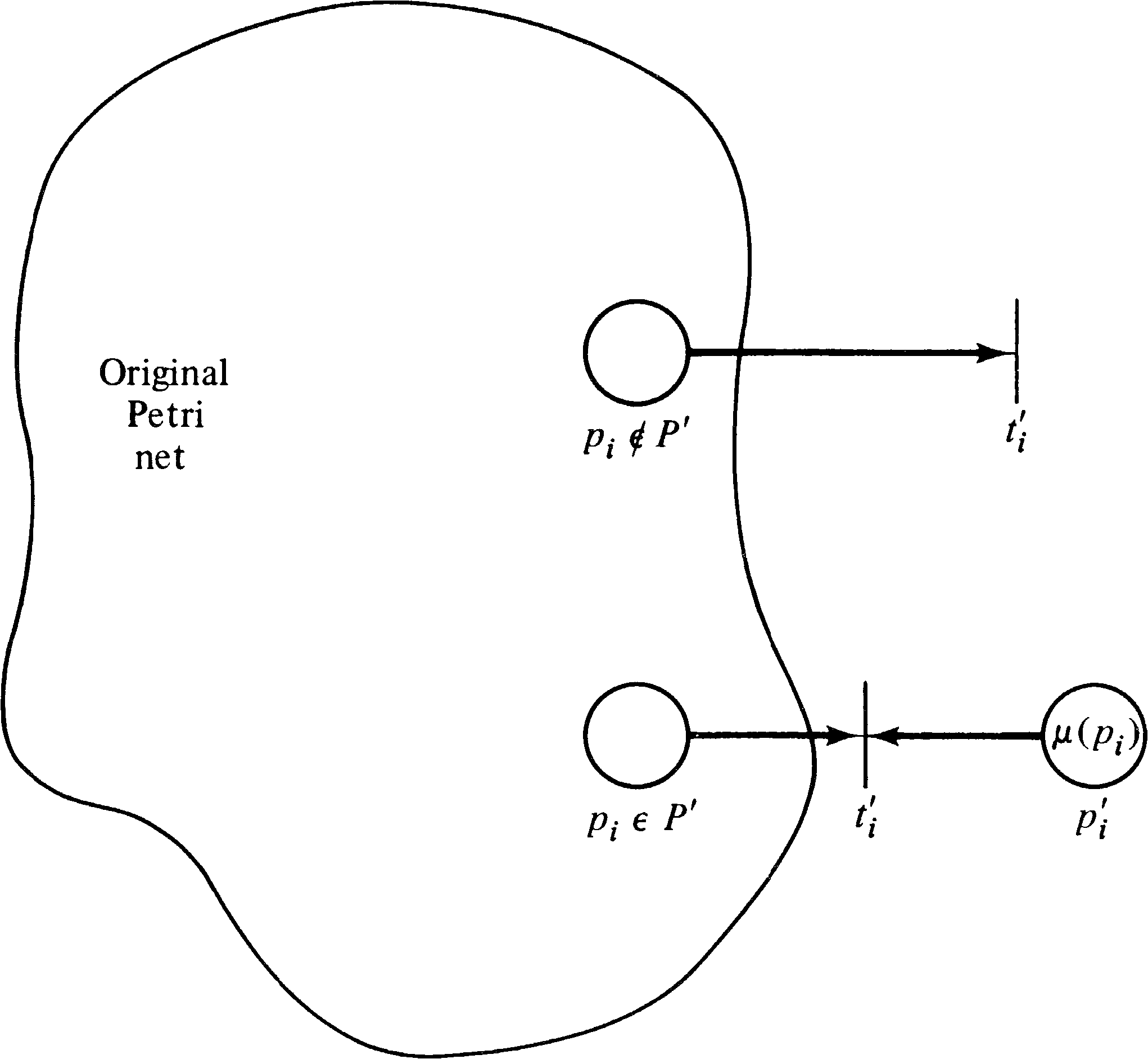Figure 5.2. A Petri net showing that the submarking reachability problem can be reduced to the zero-reachability problem. The subset of places P′ will have the marking μ in the original net if and only if the zero marking is reachable in the net as modified here.

Figure 5.2 illustrates the two types of transitions introduced. Formally we define C2 by

 P2 = P1 ∪ { pi′ | pi ∈ P′ } T2 = T1 ∪ { ti′ | pi ∈ P1 } I2(tj) = I1(tj) for tj ∈ T1 I2(ti′) = { pi } for pi \o′/∈′ P′ = { pi, pi′ } for pi ∈ P′ O2(tj) = O1(tj) for tj ∈ T1 O2(ti′) = { } for pi ∈ P1

with an initial marking

 μ2(pi) = μ1(pi), pi ∈ P1 μ2(pi′) = μ′(pi), pi ∈ P′

THEOREM 5.1
The submarking reachability problem is reducible to the zero-reachability problem.

Proof :
We show that for the Petri net C2 constructed above from C1, 0 ∈ R ( C2, μ2 ) if and only if μ′′ ∈ R ( C1, μ1 ) with μ′′ ( pi ) = μ′ ( pi ) for all piP′ .

To show that 0 ∈ R ( C2, μ2 ) if and only if there exists a μ′′ ∈ R ( C1, μ1 ) with μ′′ ( pi ) = μ′ ( pi ) for piP′, assume first that μ′′ exists in R ( C1, μ1 ) . Then in C2 we can also reach the marking μ′′ in the places piP1 by firing only those transitions from T1. Now for each piP′, we can fire ti′ exactly μ′ ( pi ) times, reducing both pi and pi′ to zero. Then we can fire ti′ for each piP′ as many times as necessary to put these to zero, so 0 ∈ R ( C2, μ2 ) .

Now assume 0 ∈ R ( C2, μ2 ) ; then there exists a sequence of transition firings σ which leads from μ2 to 0. This sequence will contain exactly μ′ ( pi ) firings of ti′ for piP′ (to remove the tokens from pi′ ) and some number of firings of ti′ for piP′ . Note that these transition firings only remove tokens from C1, and since δ ( μ′, tj ) is defined whenever δ ( μ, tj ) is defined for μ′ ≥ μ (extra tokens never hurt), the sequence σ with all ti′ firings removed is also legal and will lead to a marking μ′′ with exactly μ′ ( pi ) tokens in pi for piP′ . Thus if 0 ∈ R ( C2, μ2 ), then μ′′ ∈ R ( C1, μ1 ) with μ′′ ( pi ) = μ′ ( pi ) for piP′ . Q.E.D.

Our next task is to show that the zero-reachability problem is reducible to the single-place zero-reachability problem. The proof of this statement again involves a construction. Given a Petri net C1 = (P1, T1, I1, O1 ) with initial marking μ1, we wish to determine if 0 ∈ R ( C1, μ1 ) . We construct, from C1, a new Petri net C2 with an additional place s (P2 = P1 ∪ { s } ) such that there exists a marking μ′ ∈ R ( C2, μ2 ) with μ′ ( s ) = 0 if and only if 0 ∈ R ( C1, μ1 ) .

The construction of C2 defines s so that at all times the number of tokens in s is equal to the sum of the number of tokens in the places of C1. Thus if μ′ ( s ) = 0, then there are zero tokens in all places of C1 and vice versa. We define the initial marking μ2 by

 μ2(pi) = μ1(pi) for pi ∈ P1 μ2(s) = Σ μ′(pi) pi ∈ P1

Now for each transition tjT1, the same transition is in C2 but augmented by arcs to the place s. Define

 dj = Σ #(pi, O(tj)) − #(pi, I(tj)) pi ∈ P1

Then dj is the change in the number of tokens which results from firing transition tj. Now if dj > 0, then dj tokens must be added to place s, so we add dj arcs from tj to s; if dj < 0, then we remove − dj tokens from s by − dj arcs from s to tj.

• If dj > 0, then # ( s, I ( tj )) = 0 ; # ( s, O ( tj )) = dj.

• If dj < 0, then # ( s, I ( tj )) = − dj; # ( s, O ( tj )) = 0 .

• If dj = 0, then # ( s, I ( tj )) = 0 ; # ( s, O ( tj )) = 0 .

With this construction, any sequence of transition firings which leads C1 to the marking 0 will lead C2 to a marking μ′ with μ′ ( s ) = 0 [ μ′ ( pi ) = 0 also] and vice versa.
THEOREM 5.2
The zero-reachability problem is reducible to the single-place zero-reachability problem.

Proof :
The formal proof, based on the above construction, is left to the reader. Q.E.D.
With these two theorems, and the obvious observations, we can now conclude the following.
THEOREM 5.3
The following reachability problems are equivalent

1. The reachability problem

2. The zero-reachability problem

3. The submarking reachability problem

4. The single-place zero-reachability problem
These theorems and their proofs are mainly due to Hack [1975c].

5.3. Limited Petri Net Structures

The early work on Petri nets, and some current work, defined Petri nets in somewhat more restricted ways than the definition in Chapter 2. In particular, the following two restrictions are sometimes enforced.

RESTRICTION 5.1
The multiplicity of any place is limited to be less than or equal to 1. That is, # ( pi, I ( tj )) ≤ 1 and # ( pi, O ( tj )) ≤ 1 for all piP and tjT. This restricts the input and output bags to be sets.
RESTRICTION 5.2
No place may be both an input and an output of the same transition. I ( tj ) ∩ O ( tj ) = ∅ . This is often stated as # ( pi, I ( tj )) ⋅ # ( pi, O ( tj )) = 0, for all pi and tj.
Petri nets which satisfy Restriction 1 are called ordinary Petri nets. Petri nets which satisfy Restriction 2 are called self-loop-free Petri nets or nonreflexive Petri nets. Petri nets satisfying both restrictions are called restricted Petri nets. These classes of Petri nets are related as shown in Figure 5.3.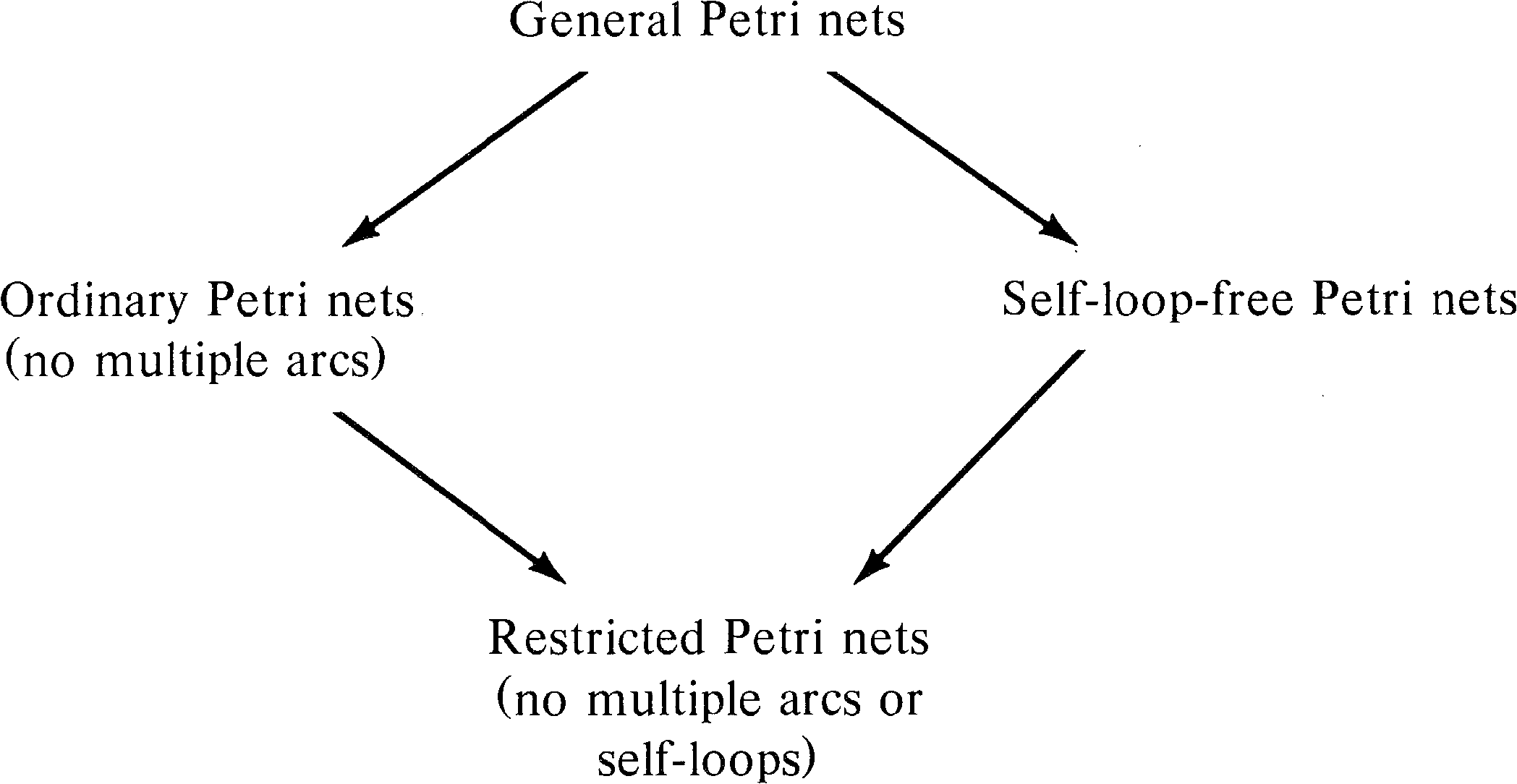Figure 5.3. The relationships among the classes of Petri nets. An arc indicates containment; reducibility arcs would be directed in the opposite direction.

These subclasses of the general Petri net model have been considered for several reasons. A major reason is that the propagation of Petri net concepts was informal in its earlier theory. The need for multiple arcs or self-loops did not occur in early modeling. Also, it was probably felt that the theory would be easier without these additional complications to the theory. As the theory has developed, however, it has become evident that the more general definitions have not been more difficult to work with. Current work using models with these restrictions is thus either the result of unnecessary timidity on the part of the researcher or the need for quicker exposition leading to simpler definitions.

However, these restrictions add nothing to our ability to analyze Petri nets. Consider the reachability problem for these classes of nets. To show the essential equivalence of these four classes of Petri nets, we prove the following.

THEOREM 5.4
The following reachability problems are equivalent.

1. General Petri nets

2. Ordinary Petri nets

3. Self-loop-free Petri nets

4. Restricted Petri nets

Proof :
The following reducibilities are obvious from the definitions.

1. The reachability problem for ordinary Petri nets is reducible to the reachability problem for general Petri nets.

2. The reachability problem for self-loop-free Petri nets is reducible to the reachability problem for general Petri nets.

3. The reachability problem for restricted Petri nets is reducible to both the reachability problem for ordinary Petri nets and the reachability problem for self-loop-free Petri nets.

We show that general Petri nets can be transformed into restricted Petri nets in such a way as to reduce the reachability problem for general Petri nets to the reachability problem for restricted Petri nets. This then shows that these four reachability problems are equivalent.

To transform a general Petri net into a restricted Petri net, we use the following basic approach. Every place in the general Petri net is replaced by a ring of places in the restricted Petri net. Figure 5.4 shows the general form of a ring of places. Notice that a collection of tokens placed in the ring can freely move around the ring to any position at any time; they can all group into place pi,1 or spread out uniformly to cover all ki places in the ring. Thus a transition which needs three tokens from place pi can pick up one from each of pi,1, pi,2, and pi,3 rather than all three from pi. Similarly a transition which uses pi both as an input and as an output (a self-loop) may input from pi,1 and output to pi,2, eliminating the self-loop.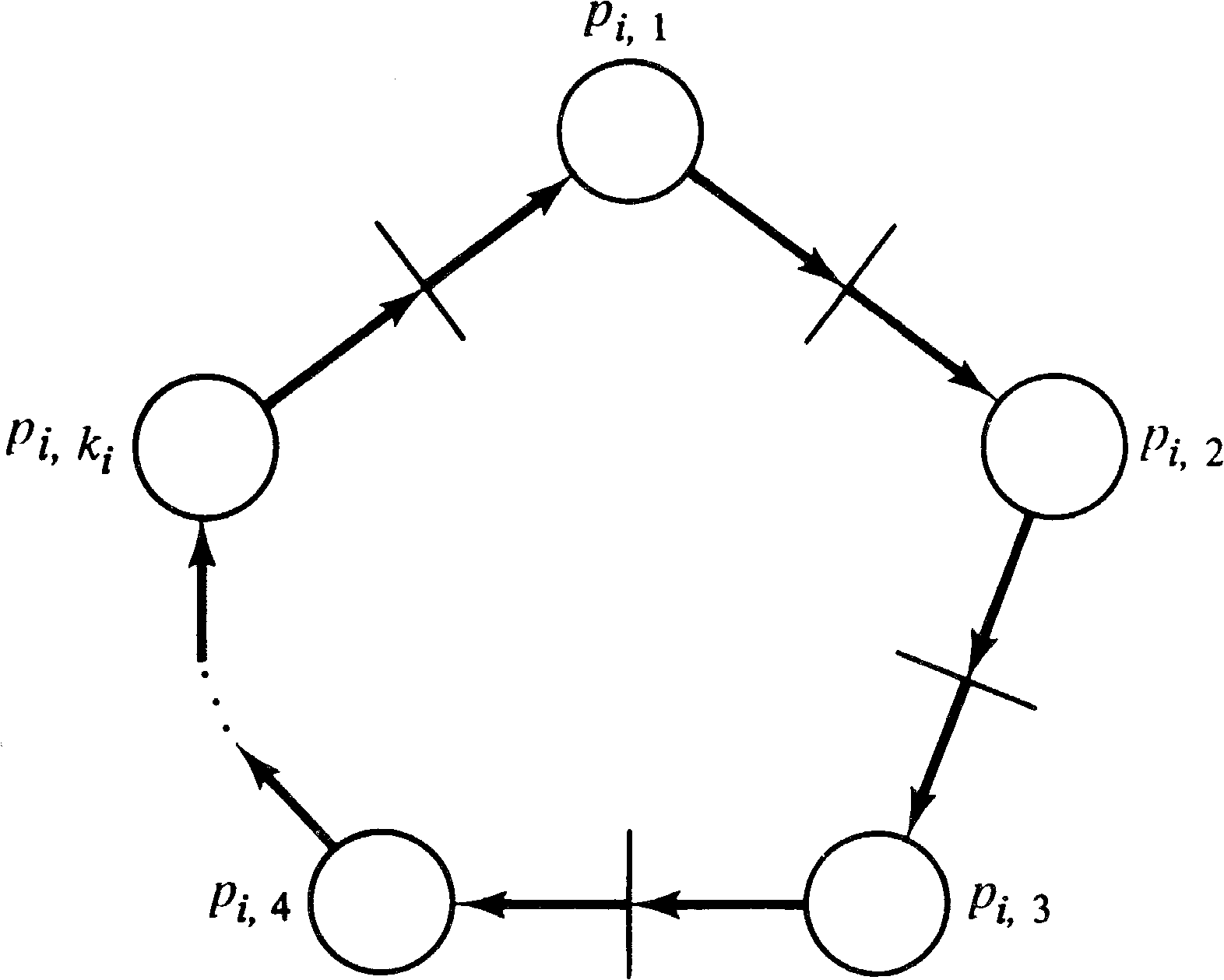Figure 5.4. A ring of places to be used in a restricted Petri net to represent a place in a general Petri net. The number of places ki representing a place pi is determined by the sum of the maximum multiplicities of the place.

Formally, for a general Petri net C1 = (P1, T1, I1, O1 ) with marking μ1, we define a restricted Petri net C2 = (P2, T2, I2, O2 ) with marking μ2 as follows. First define, for each piP1, an integer ki by

 ki = max (#(pi, I(tj)) + #(pi, O(tj))) tj ∈ T1

The restricted Petri net C2 is defined by

 P2 = { p i, h | pi ∈ P1, 1 ≤ h ≤ ki } T2 = T1 ∪ { t i, h | p i, h ∈ P2 }

The input and output functions for “normal” transitions are defined such that

 #(p i, h , I2(tj)) = 1 if 1 ≤ h ≤ #(pi, I1(tj)) = 0 otherwise #(p i, h , O2(tj)) = 1 if #(pi, I1(tj)) < h ≤ #(pi, I1(tj)) + #(pi, O1(tj)) = 0 otherwise

while for the “ring” transitions,

 I2(t i, h ) = { p i, h } O2(t i, h ) = { p i, n | n = 1 + (h mod ki) }

The marking μ2 is defined by

 μ2(pi,1) = μ1(pi) for pi ∈ P1 μ2(p i, h ) = 0 for h > 1

By construction, for any marking μ which is reachable in C1, there exists a marking μ′ of C2 such that

 Σ μ′(p i, h ) = μ(pi) for all pi ∈ P1 h

In particular it is possible to move all tokens from p i, h to pi,1 in C2 at any time. Thus, we can define a marking μ′ by

 μ′(pi,1) = μ(pi) for pi ∈ P1 μ′(p i, h ) = 0 for h > 1

and μ′ is reachable in the restricted Petri net C2 if and only if μ is reachable in C1. Q.E.D.

Thus, from the point of view of analysis, general Petri nets and these three restricted classes of the general Petri net -- ordinary Petri nets, self-loop-free Petri nets, and restricted Petri nets -- are equivalent, each can be transformed into a similar net of another class, allowing a reachability problem in one to be reduced to a reachability problem in another. The constructions in this section are due to Hack [1974a].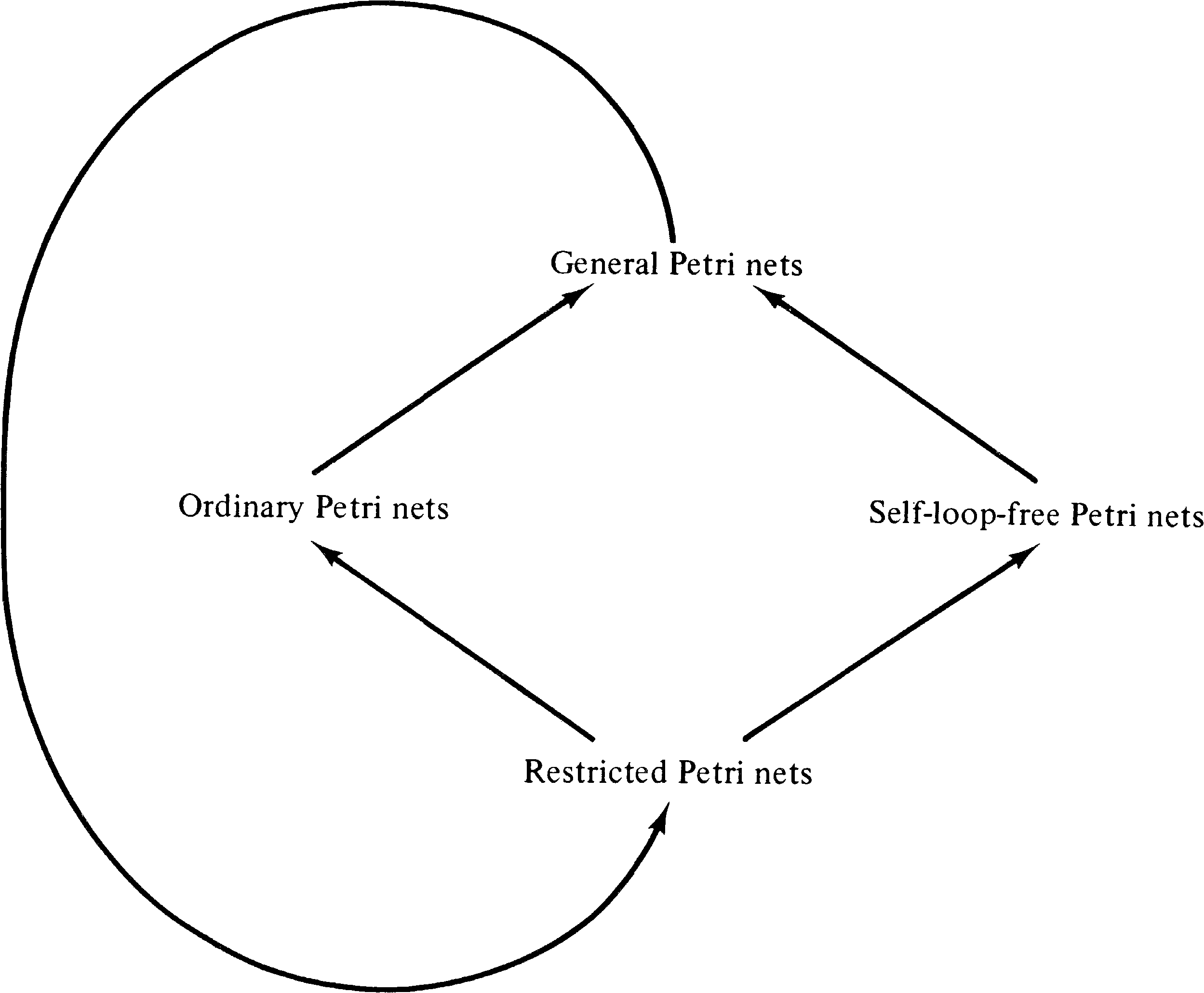Figure 5.5. Reducibility of the reachability problem among classes of limited Petri nets.

5.4. Liveness and Reachability

Reachability is an important problem, but not the only remaining problem for Petri nets. Liveness is another problem which has received much attention in the Petri net literature. As pointed out in Section 4.1.4, liveness is related to deadlock. Two liveness problems for a Petri net C = (P, T, I, O) with initial marking μ are of concern here. A Petri net is live if each transition is live. A transition tj is live in a marking μ if for each μ′ ∈ R ( C, μ ) there exists a sequence σ such that tj is enabled in δ ( μ′, σ ) . A transition tj is dead in a marking μ if there is no reachable marking in which it can fire.

DEFINITION 5.7 Liveness Problem
For all transitions tjT, is tj live?
DEFINITION 5.8 Single-Transition Liveness Problem
Given tjT, is tj live?

The liveness problem is obviously reducible to the single-transition liveness problem. To solve the liveness problem, we simply solve the single-transition liveness problem for each tjT; if | T | = m, then we must solve m single-transition liveness problems.

The reachability problem can also be reduced to the liveness problem. Since the many variants of the reachability problem are equivalent, we use the single-place zero-reachability problem. If we have any of the other reachability problems, they can be reduced to the single-place zero-reachability problem as shown in Section 5.2. Now, if we wish to determine if place pi can be zero in any reachable marking for a Petri net C1 = (P1, T1, I1, O1 ) with initial marking μ1, we construct a Petri net C2 = (P2, T2, I2, O2 ) with initial marking μ2, which is live if and only if the zero marking is not reachable from μ1.

The Petri net C2 is constructed from C1 by the addition of two places, r1 and r2, and three transitions, s1, s2, and s3. We first modify all transitions of T1 to include r1 as both an input and an output. The initial marking μ2 will include a token in r1. The place r1 is a “run” place; as long as the token remains in r1 the transitions of T1 can fire normally. Thus any marking which is reachable in the places of P1 in C1 is also reachable in C2. Transition s1 is defined to have r1 as its input and a null output. This allows the token in r1 to be removed, disabling all transitions in T1 and “freezing” the marking of P1. (Note that all transitions of T1 are in conflict and, by construction if not by definition, that no more than one transition can fire at a time.)

The place r1 and transition s1 allow the net C1 to reach any reachable marking and then for s1 to fire and freeze the net at that marking. Now we need to see if place pi is zero. We introduce a new place r2 and a transition s2 which has pi as its input and r2 as its output. If pi can ever become zero, this transition is not live; in fact the entire net is dead if transition s1 fires in that marking. Hence if pi can be zero, the net is not live. If pi cannot be zero, then s2 can always fire, putting a token in r2. In this case we must put a token back in r1 and assure that all transitions in C2 are live. We must be sure that C2 is live even if C1 is not live. This is accomplished by a transition s3 which “floods” the net C2 with tokens, assuring that every transition is live if a token is ever put in r2. Transition s3 has r2 as its input and every place of C2 (all pi in C1 and r1 and r2 ) as output. This construction is illustrated in Figure 5.6.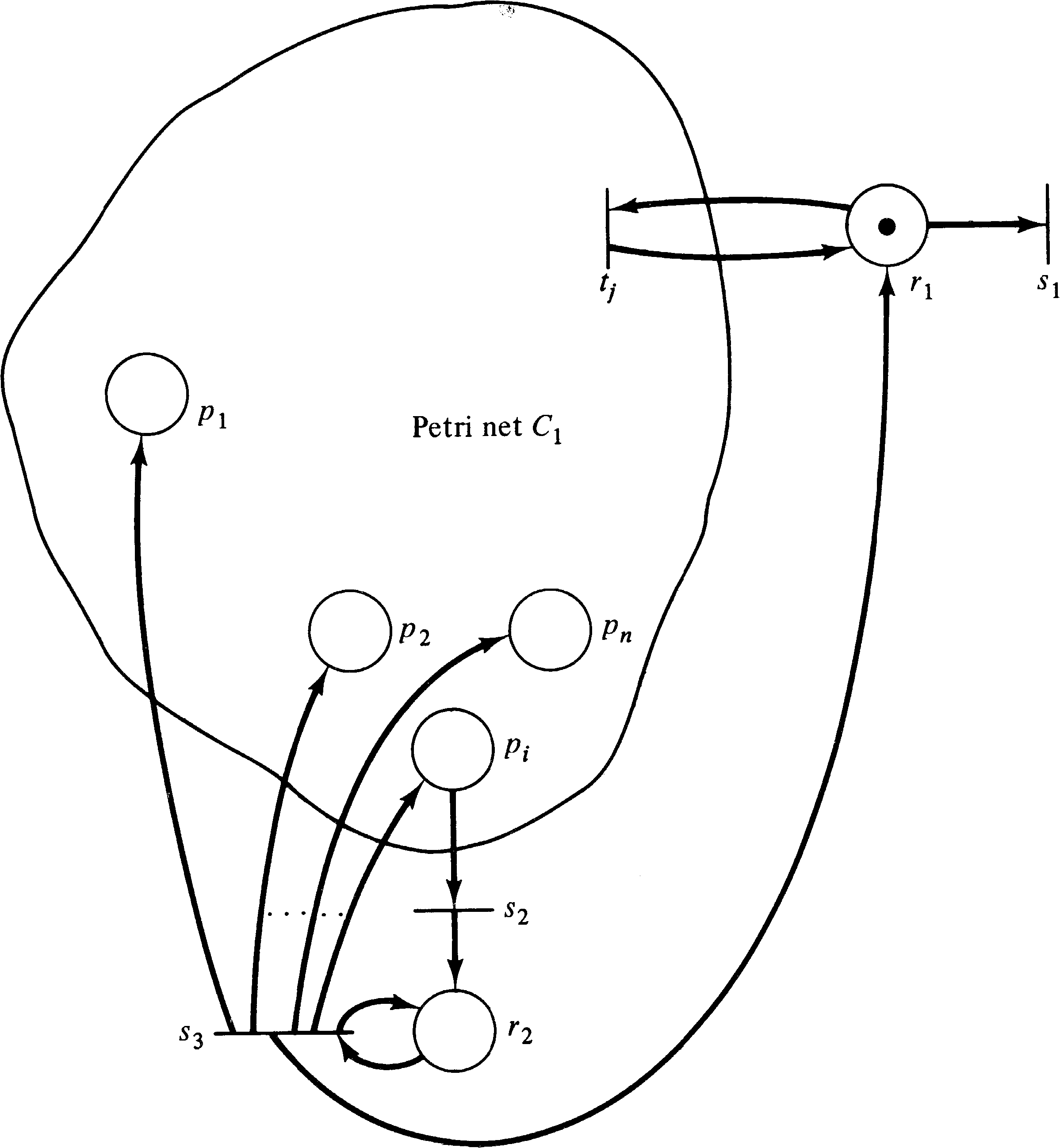Figure 5.6. A construction converting the single-place zero-reachability problem [is a marking reachable with μ ( pi ) = 0 ?] to the liveness problem [is this net live?].

Now, if a marking μ is reachable in R ( C1, μ1 ) with μ ( pi ) = 0, then the net C2 can also reach this marking on the place of P1 by executing the same sequence of transition firings. Then s1 can fire, freezing the C1 subset. Since μ ( pi ) = 0, transition s2 cannot fire and C2 is dead. Thus if pi can become zero, then C2 is not live.

Conversely, if C2 is not live then, a marking μ must be reachable in which μ ( r2 ) = 0 and there is no reachable state in which r2 has a token. [If r2 has a token, s3 is enabled, and s3 can be fired repeatedly enough times to enable any (or all) transitions, and so the net is live.] If r2 has no token and cannot get any, then the marking of pi must also be zero. Thus if C2 is not live, then a marking is reachable in which the marking of pi is zero.

On the basis of this construction, we have the following.

THEOREM 5.5
The reachability problem is reducible to the liveness problem.

Now we need to show the following.

THEOREM 5.6
The single-transition liveness problem is reducible to the reachability problem.

The proof that the single-transition liveness problem is reducible to the reachability problem rests on testing for the reachability of any of a finite set of maximal tj -dead submarkings. A Petri net is not live for a transition tj if and only if any marking is reachable in which the transition tj is not fireable and cannot become fireable. A marking of this sort is called tj -dead. For any marking μ we can test if it is tj -dead by constructing the reachability tree with μ as the root and testing if transition tj can fire anywhere in the tree. If it cannot then μ is tj -dead. Checking for liveness of tj then requires checking if any tj -dead marking is reachable.

In general, however, there may be an infinite number of tj -dead markings and an infinite set of markings in which to find the tj -dead markings. The set of markings which must be checked for reachability is reduced to a finite number by noting two properties. First, if a marking μ is tj -dead, then any marking μ′ ≤ μ is also tj -dead. (Any firing sequence possible from μ′ is also possible from μ, so if μ′ could lead to the firing of tj, so could μ .) Second, the markings of some places will not affect the tj -deadness of a marking, and so the markings of these places are “don't-cares”; they can be arbitrary. Borrowing from the reachability tree construction, we replace these “don't-care” components by ω to indicate that an arbitrarily large number of tokens can be in this place without affecting the tj -deadness of the marking. Now since any μ′ ≤ μ is tj -dead if μ is tj -dead, we need not consider those places pi with μ ( pi ) = ω . This means we use the submarking reachability problem with P′ = { pi | μ ( pi ) ≠ ω } .

As an example, consider the Petri net of Figure 5.7. The markings (2, 0), (1, 0), (0, 0), (0, 1), (0, 2), (0, 3), … are tj -dead, but they can be finitely represented by the set { (0, ω ), (2, 0), (1, 0) } .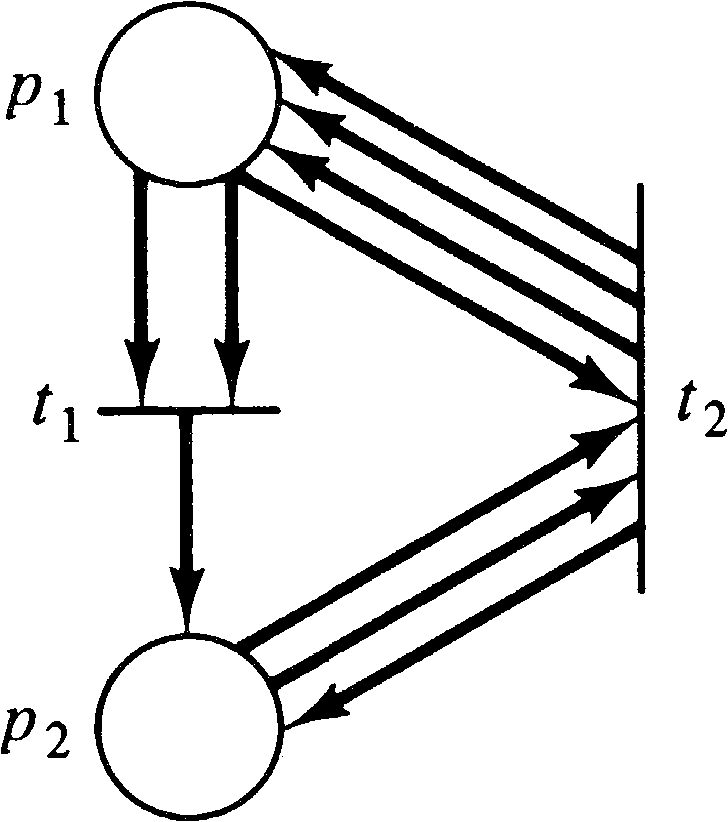Figure 5.7. A Petri net to illustrate tj -dead markings.

Hack [1974c; 1975c] has shown that there exists for a Petri net C a finite set Dt of markings (extended to include ω ) such that C is live if and only if no marking in Dt is reachable. If a marking of Dt contains ω, submarking reachability is implied.

Further, Dt can be effectively computed. Since Dt is finite, the non- ω -components of the markings have an upper bound b. This bound b is characterized as the smallest number such that for any marking μ with μ ( pi ) ≤ b + 1 for all pi, if μ is tj -dead, then the submarking μ′, with μ′ ( pi ) = μ ( pi ) if μ ( pi ) ≤ b and μ′ ( pi ) = ω if μ ( pi ) = b + 1, is tj -dead. With this characterization of b, we can construct Dt as follows.

1. Compute b. Start with b = 0, and increase b until the first b is found which satisfies the characterization of the bound defined above. Testing for b requires checking all ( b + 2 )n markings with components less than or equal to b + 1 .

2. Compute Dt by testing all markings and submarkings with components less than or equal to b or equal to ω . Dt is the set of tj -dead markings from this set of ( b + 2 )n -vectors.

Once we have constructed Dt, we then apply the submarking reachability problem for each element of Dt. If any element of Dt is reachable from the initial marking, the Petri net is not live; if no element of Dt is reachable, the Petri net is live.

From these two theorems, we have the following.

THEOREM 5.7
The following problems are equivalent:

1. The reachability problem

2. The liveness problem

3. The single-transition liveness problem

More formal proofs of the reducibility of liveness to reachability can be found in [Hack 1974c; Hack 1975c].

5.5. Undecidable Results

In Section 5.4 we have shown that a number of problems in reachability and liveness are equivalent, but no result has been obtained yet on the decidability of these problems. To show decidability, it is necessary to reduce a Petri net problem to a problem with a known solution, or to show undecidability, to reduce a problem which is known to be undecidable to a Petri net problem. The first important result of this kind was by Rabin [Baker 1973b]. Rabin showed that for two Petri nets C1 with marking μ1 and C2 with marking μ2 it is undecidable if R ( C1, μ1 ) ⊆ R ( C2, μ2 ) . Hack [1975a] later strengthened this to show that it is undecidable if R ( C1, μ1 ) = R ( C2, μ2 ) . The proof of these statements is based on Hilbert's tenth problem. (In 1900, D. Hilbert presented 23 problems to a conference of mathematicians; this was the tenth in his list.)

DEFINITION 5.9 Hilbert's Tenth Problem
Given a polynomial P over n variables with integer coefficients, does there exist a vector of integers, ( x1, x2, …, xn ) such that

 P(x1, x2, …, xn) = 0?

The equation P ( x1, x2, …, xn ) = 0 is a Diophantine equation. In general it will be a sum of terms

 P(x1, x2, …, xn) = Σ Ri(x1, x2, …, xn) i Ri(x1, x2, …, xn) = ai ⋅ x s1 ⋅ x s2 ⋅ ⋯ ⋅ x sh

Diophantine equations include x1 = 0, 3 x1x2 + 6 x3 = 0, and so on.

In 1970, Matijasevic proved that Hilbert's tenth problem was undecidable [Davis 1973; Davis and Hersh 1973]: There is no general algorithm to determine if an arbitrary Diophantine equation has a root (a set of values for which the polynomial is zero). This forms the basis of the proof that the equality problem for Petri net reachability sets is undecidable. The strategy is to construct for a Diophantine polynomial a Petri net which (in some sense) computes all values of the polynomial.

5.5.1. The Polynomial Graph Inclusion Problem

The proof of the undecidability of the equality problem is in three parts (Figure 5.8). First, Hilbert's tenth problem is reduced to the polynomial graph inclusion problem. Then the polynomial graph inclusion problem is reduced to the subset problem for Petri net reachability sets. Finally, the subset problem for Petri net reachability sets is reduced to the equality problem for Petri net reachability sets. This shows that Hilbert's tenth problem, known to be undecidable, is reducible to the equality problem, which must therefore also be undecidable.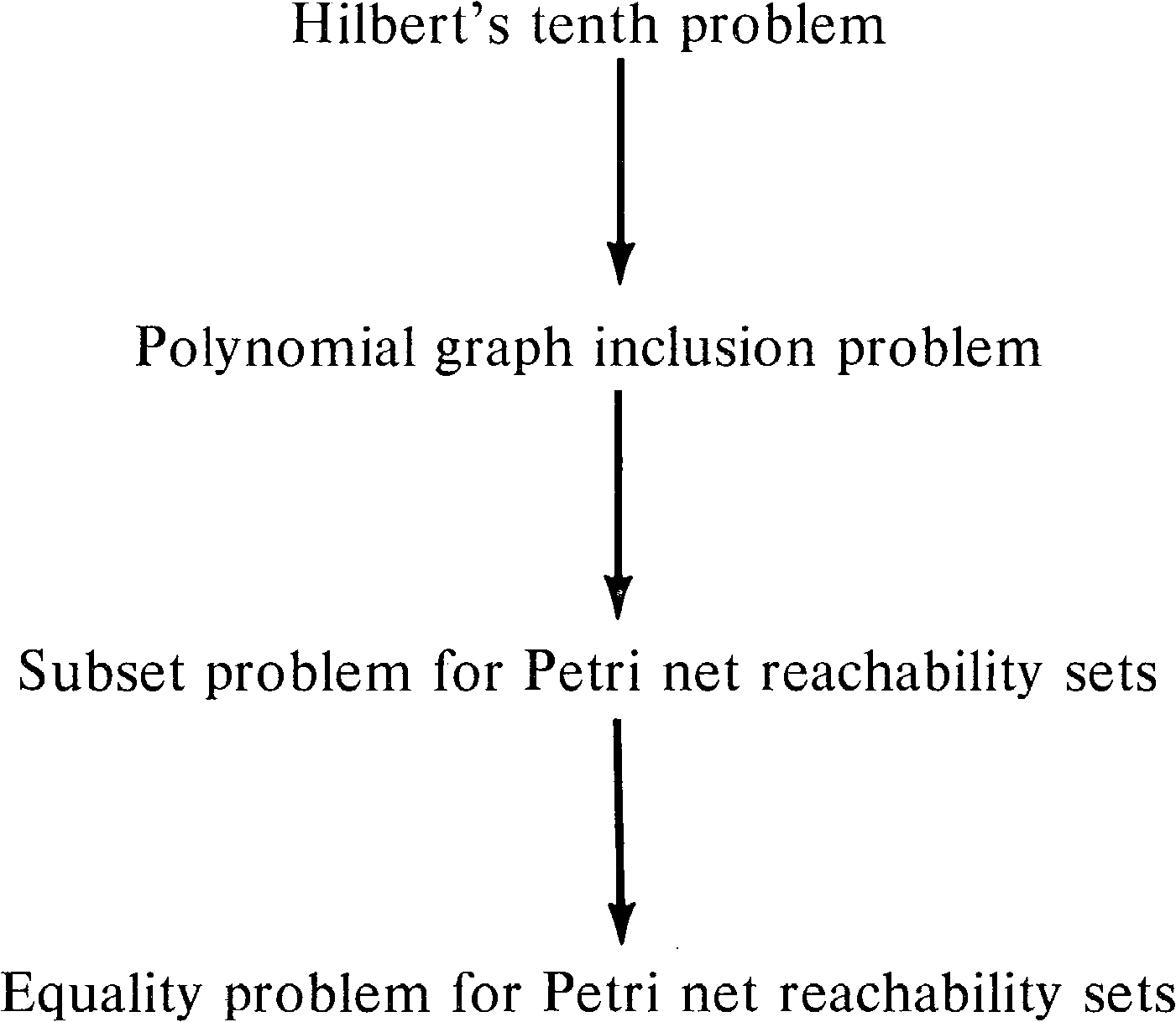Figure 5.8. The reducibilities showing that the equality (and subset) problem for Petri net reachability sets is undecidable.

DEFINITION 5.10
The graph G ( P ) of a Diophantine polynomial P ( x1, …, xn ) with nonnegative coefficients is the set

 G(P) = { (x1, …, xn, y) | y ≤ P(x1, …, xn) with 0 ≤ x1, …, xn, y }

DEFINITION 5.11
The polynomial graph inclusion problem is to determine for two Diophantine polynomials A and B if G ( A ) ⊆ G ( B ) .
We first show that Hilbert's tenth problem is reducible to the polynomial graph inclusion problem. This proves the following.
THEOREM 5.8
The polynomial graph inclusion problem is undecidable.

Proof :

1. We limit our proof to problems with nonnegative solutions. If ( x1, …, xn ) is a solution to P ( x1, …, xn ) = 0, with xi < 0, then ( x1, …, − xi, …, xn ) is a solution to P ( x1, …, − xi, …, xn ) = 0 . Thus, for an arbitrary polynomial, we need only test each of the 2n polynomials which result from changing the sign of some subset of variables for nonnegative solutions to determine the solution for the arbitrary polynomial.

2. Similarly, since P2( x1, …, xn ) = 0 if and only if P ( x1, x2, …, xn ) = 0, we need only consider polynomials whose value is nonnegative.

3. Now we can separate any polynomial P ( x1, x2, …, xn ) into two polynomials Q1( x1, …, xn ) and Q2( x1, …, xn ) such that P ( x1, …, xn ) = Q1( x1, x2, …, xn ) − Q2( x1, …, xn ) by putting all terms with positive coefficients in Q1 and all terms with negative coefficients in Q2. Now since P ( x1, …, xn ) ≥ 0 (by 2 above), we have Q1( x1, …, xn ) ≥ Q2( x1, …, xn ) and P ( x1, …, xn ) = 0 if and only if Q1( x1, x2, …, xn ) = Q2( x1, …, xn ) .

4. Consider the two polynomial graphs

 G(Q1) = { (x1, …, xn, y) | y ≤ Q1(x1, …, xn) } G(Q2 + 1) = { (x1, …, xn, y) | y ≤ 1 + Q2(x1, …, xn) }

Now, G ( Q2 + 1) ⊆ G ( Q1 ) if and only if for all nonnegative x1, …, xn and y, y ≤ 1 + Q2( x1, …, xn ) implies that yQ1( x1, …, xn ) . This is true if and only if there does not exist x1, …, xn and y such that

 Q1(x1, …, xn) < y ≤ 1 + Q2(x1, …, xn)

But from 3 above, Q1Q2 so that

 Q1(x1, …, xn) < y ≤ 1 + Q2(x1, …, xn) ≤ 1 + Q1(x1, …, xn)

and, since all quantities are integers,

 y = 1 + Q2(x1, …, xn) = 1 + Q1(x1, …, xn)

which is true if and only if Q1 = Q2. Thus, we see that G ( Q2 + 1) ⊆ G ( Q1 ) if and only if there does not exist x1, …, xn such that Q1( x1, …, xn ) = Q2( x1, …, xn ), which is to say there does not exist x1, …, xn such that P ( x1, …, xn ) = 0 .

5. Therefore to determine that the equation P ( x1, x2, …, xn ) = 0 has a solution, we need only to show that it is not the case that G ( Q2 + 1) ⊆ G ( Q1 ) .
Q.E.D.

5.5.2. Weak Computation

Now we need to show that Petri nets can (in some sense) compute the value of a polynomial Q ( x1, x2, …, xn ) . We have carefully limited the polynomial Q to having a nonnegative value, nonnegative coefficients, and nonnegative variables. This allows us to encode the values of the variables and the value of the polynomial as the number of tokens in places in a Petri net. Figure 5.9 shows the general scheme. The input values x1, …, xn are encoded by xi tokens in pi for i = 1, …, n. Initially a token also resides in the “run” place. The execution of the net will terminate by placing a token in the “quit” place. At this time the “output” place will have y tokens, where yQ ( x1, …, xn ) .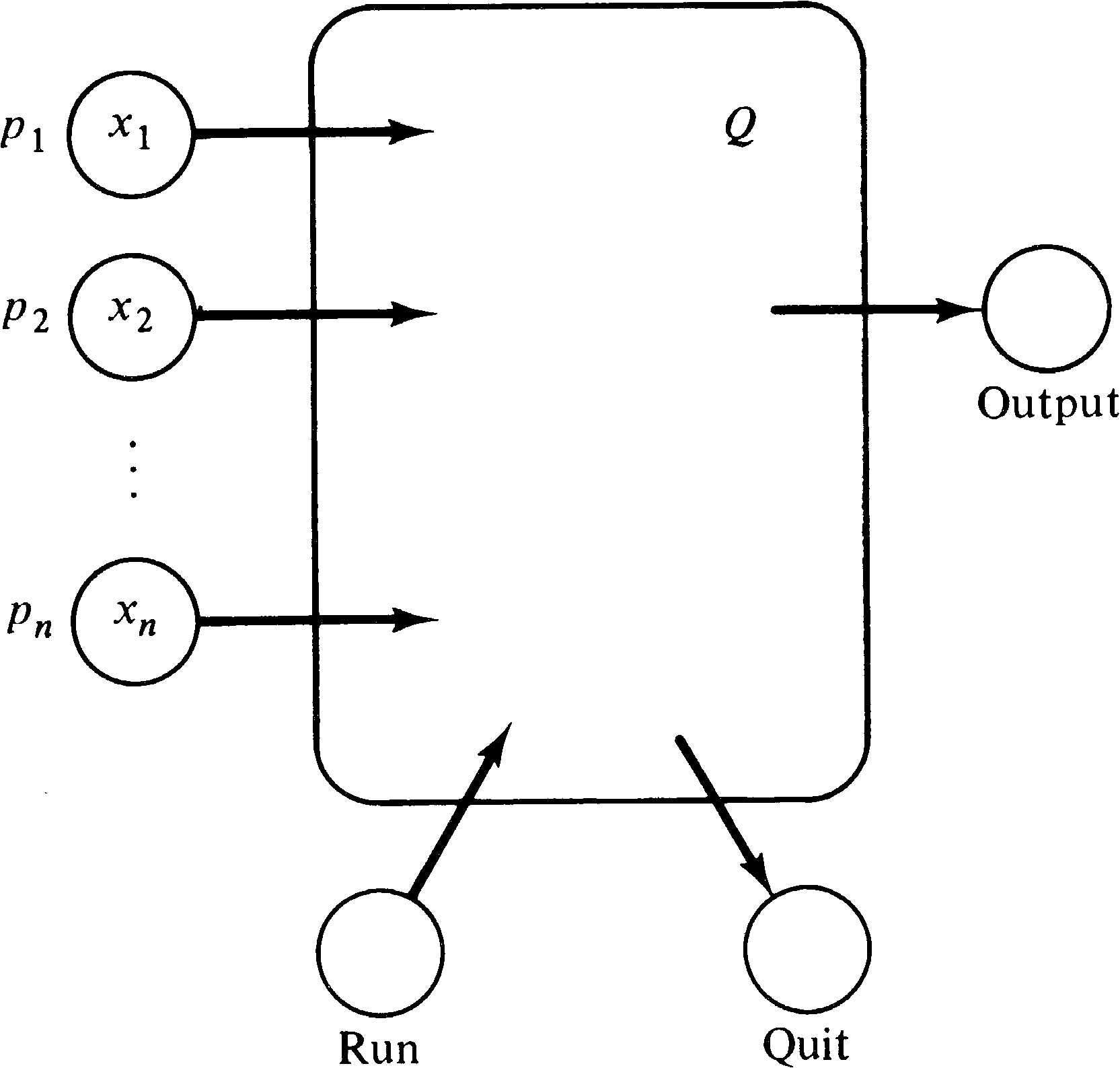Figure 5.9. Basic structure of a Petri net to weakly compute the value of a polynomial, Q ( x1, x2, …, xn ) .

This Petri net will weakly compute the value Q ( x1, …, xn ) . Weak computation means that the value computed will not exceed Q ( x1, …, xn ) but may be any (nonnegative) value less than Q ( x1, …, xn ) . Weak computation is necessary for Petri nets because of the permissive nature of transition firings; a Petri net cannot be forced to finish. The definition of a polynomial graph G ( Q ) was made specifically with this in mind.

What we show now is that subnets can be constructed which weakly compute the function of (binary) multiplication. From this, we can construct a composite net which weakly computes the value of each term of a polynomial by successive multiplication subnets. The output of the subnet for each term will be deposited in the output place for the polynomial. Thus the number of tokens in the output place will be the sum of the outputs for each term.

The multiplication subnet is shown in Figure 5.10. This net will weakly compute the product of the numbers, x and y, of tokens in its two inputs and place this many tokens in its output. The operation of the net is quite simple. To compute the product of x and y, transition t1 first fires, moving one token from px to p2. This token enables transition t3, which can now copy y tokens from place py, putting them in p3 and putting y tokens in p xy , the output place. Now t2 can fire, putting the token in p2 back into p1. This enables t4, which can copy the y tokens from p3 back into py. This entire process can be repeated exactly x times, each time putting y tokens in p xy . Then the marking of place px has been reduced to zero, and the net must stop. The total number of tokens in place p xy is then the product of x and y.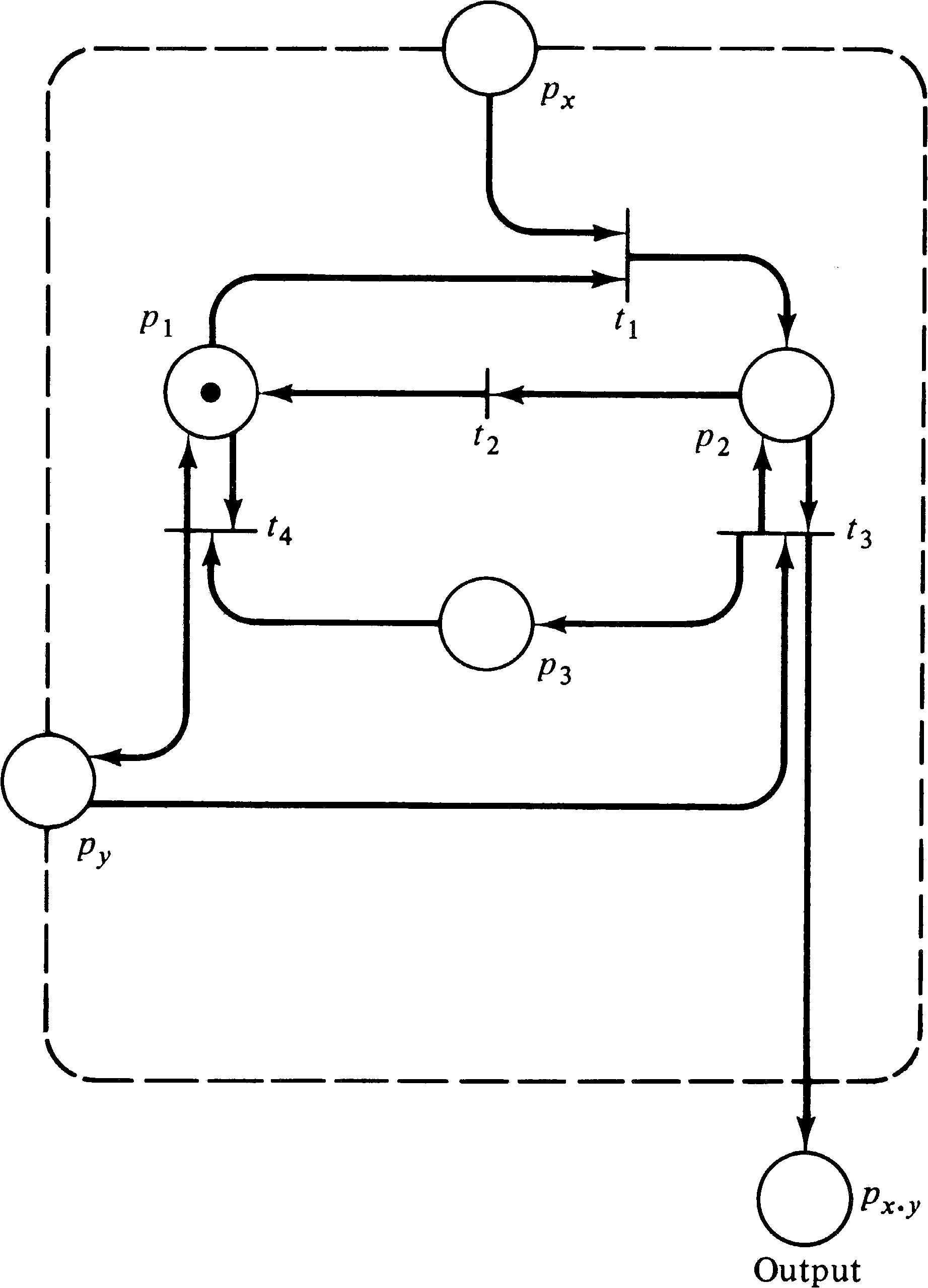Figure 5.10. A multiplier subnet. This subnet weakly computes the product of x and y.

The above case is the best case, in the sense that the number of output tokens is exactly xy. However, the token in p2 enables both transitions t3 and t2, and it is possible for t2 to fire before all y tokens have been copied from py to p3 and been added to p xy . In this case, the number of tokens deposited in p xy will be less than xy. Since t3 can fire no more than y times for each firing of t1 and t1 can fire no more than x times, we can guarantee that the number of tokens in p xy never exceeds xy, but because of the permissive nature of transition firings, we cannot guarantee that the number of tokens in p xy will actually equal xy; it could be less. Thus, this Petri net weakly computes the product of x and y. Now to weakly compute a term Ri which is the product ai x s1 x s2... x sh we construct a Petri net of the form shown in Figure 5.11. Since each subnet weakly computes the product of two terms, the entire subnet weakly computes the value of the term Ri.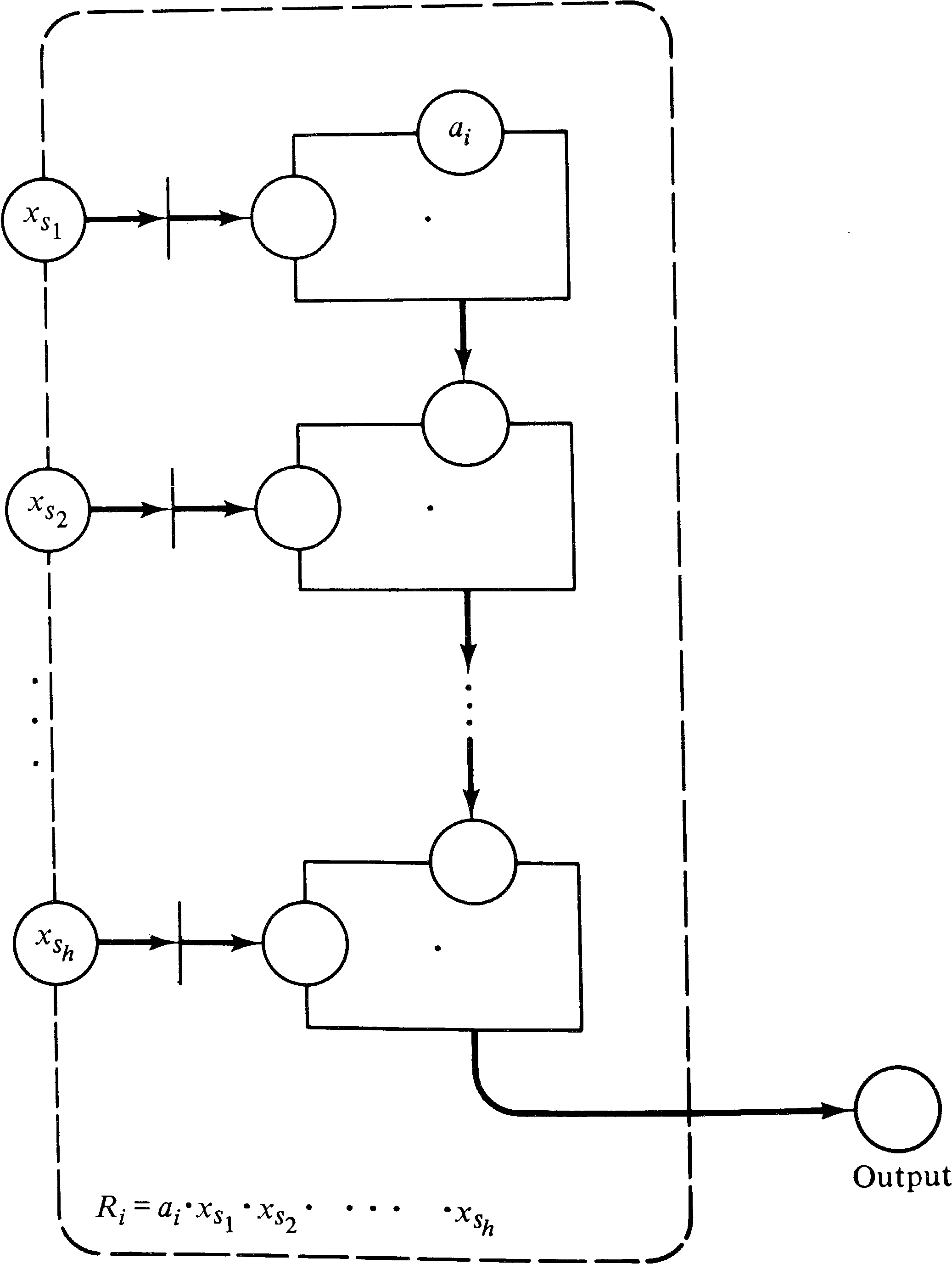Figure 5.11. A Petri net structure to weakly compute a term of a Diophantine polynomial. Each box is a net of the form of Figure 5.10.

Figure 5.12 then shows how a polynomial P = R1 + R2 + ⋯ + Rk can be weakly computed. Each subnet is of the form of Figure 5.11 and weakly computes the value of one term. The outputs of the k subnets for each term have been merged together, giving a total value which is the sum of each term.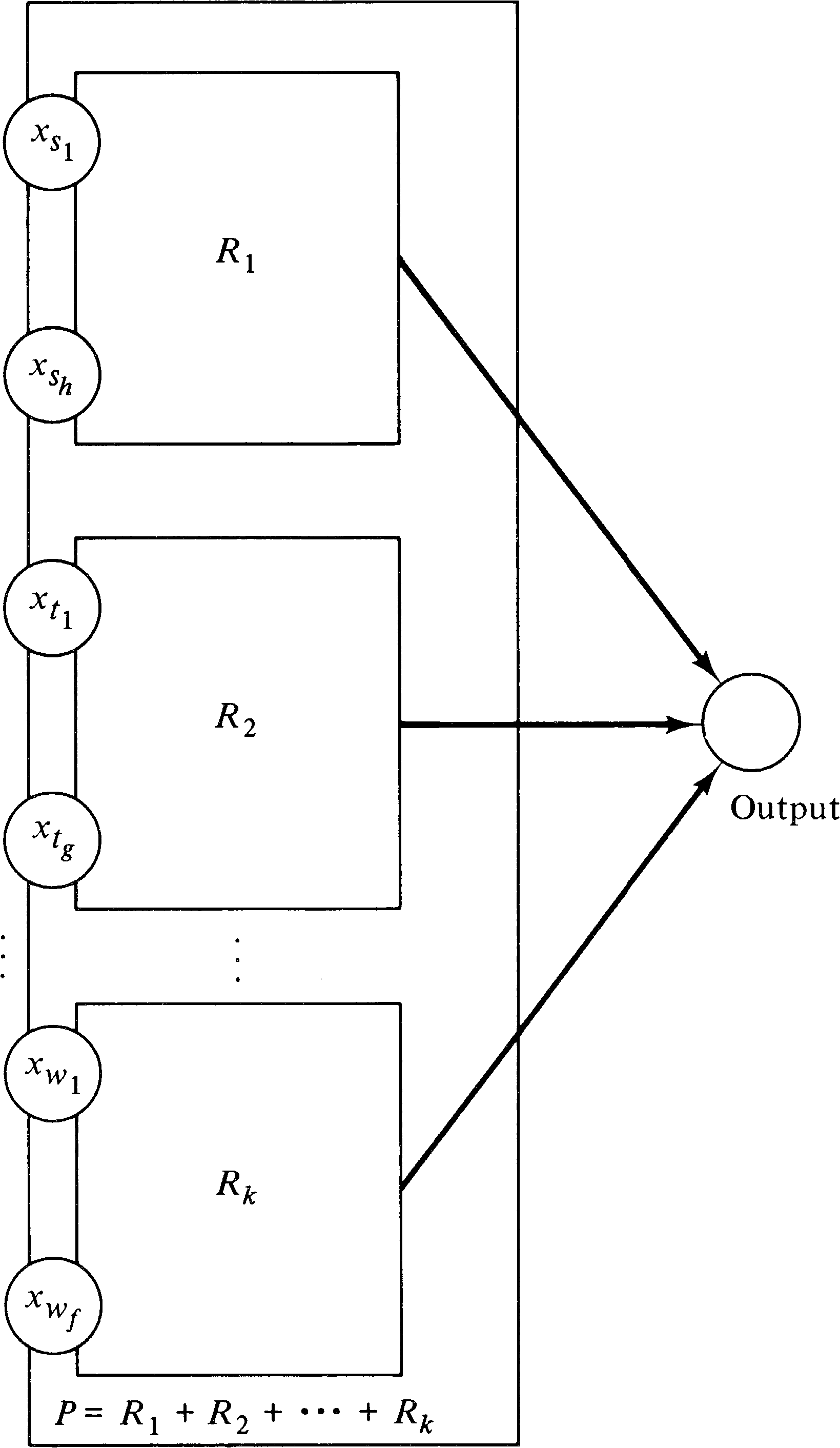Figure 5.12. A Petri net to weakly compute P ( x1, x2, …, xn ) by using a collection of subnets of the form in Figure 5.11.

Now some control transitions and places are added to create the specific reachability sets needed. First we need to be able to produce an arbitrary value for each of the variables ( x1, …, xn ) and record that value in the places p1, …, pn. A transition ti is created for each pi with null input and outputs to pi and every place which is an input corresponding to xi in a term Rj which uses xi. Thus, in the polynomial x1 + x1 x2 we would have a transition t1 with outputs to p1 and to the x1 inputs of the two terms, x1 and x1 x2, which use x1; t2 would output to p2 and to the x2 input of the term x1 x2.

These transitions can fire an arbitrary number of times, creating any value in p1, …, pn. Thus, for every yP ( x1, …, xn ) a marking μ is reachable with μ ( p1 ) = x1, …, μ ( pn ) = xn and μ ( output ) = y. The value y = P ( x1, …, xn ) can be achieved by first firing t1 x1 times, putting x1 tokens in p1, then firing t2 x2 times, and so on until tn has fired xn times. The subnet for each term Ri of the polynomial can then execute, with the resulting polynomial value put in the output place.

To reduce the polynomial graph inclusion problem to the subset problem for Petri net reachability sets, we perform the following steps. For polynomials A and B, we wish to determine if G ( A ) ⊆ G ( B ) .

1. We construct the Petri net CA which weakly computes A ( x1, …, xn ) and the Petri net CB which weakly computes B ( x1, …, xn ) .

2. If the number of places in the two nets is not equal, we add places to the smaller to equalize the number of places. These places are initially unmarked and are not used by any of the transitions in the net.

3. Now we must eliminate the effects of all internal places on the reachability sets. A set of n + 1 places are distinguished in both CA and CB, the places corresponding to the values of x1, …, xn and the output of each net. All other places are internal places, whose markings are unimportant. However, we may find that for an internal place pi in CA and corresponding pi′ in CB that there exists a marking μ in R ( CA, μA ) with no equal marking μ′ in R ( CB, μB ), because μ ( pi ) ≠ μ′′ ( pi ) for all μ′′ in R ( CB, μB ) .

To prevent this problem we add two new places q and r to CA (giving CA′ ) and q′ and r′ to CB (giving CB′ ). In CA′, q, and r are not used for any transitions, and initially r is empty and q is marked with one token. In CB′, r′ is a “run” place. It is initially marked, and every transition in CB′ is modified to include r′ as both an input and an output. Thus, as long as the token remains in r′, the net CB′ can function as before. A new transition transfers the enabling token from r′ to q′, disabling all transitions in CB′ and “freezing” the marking. Now we add two new transitions for each internal place in CB′ .

For each internal place pi whose marking is unimportant, one transition has places q′ and pi as inputs and only q′ as an output (allowing the marking in pi to be decreased by one), and another transition has q′ as input and both q′ and pi as outputs (allowing the marking in pi to be increased by one). These transitions allow the marking of each internal place to be made arbitrary by an appropriate sequence of increasing or decreasing firings.

4. The new construction is illustrated in Figure 5.13. For these two Petri nets, CA′ and CB′ with initial marking μA′ and μB′, respectively, G ( A ) ⊆ G ( B ) if and only if R ( CA′, μA′ ) ⊆ R ( CB′, μB′ ) .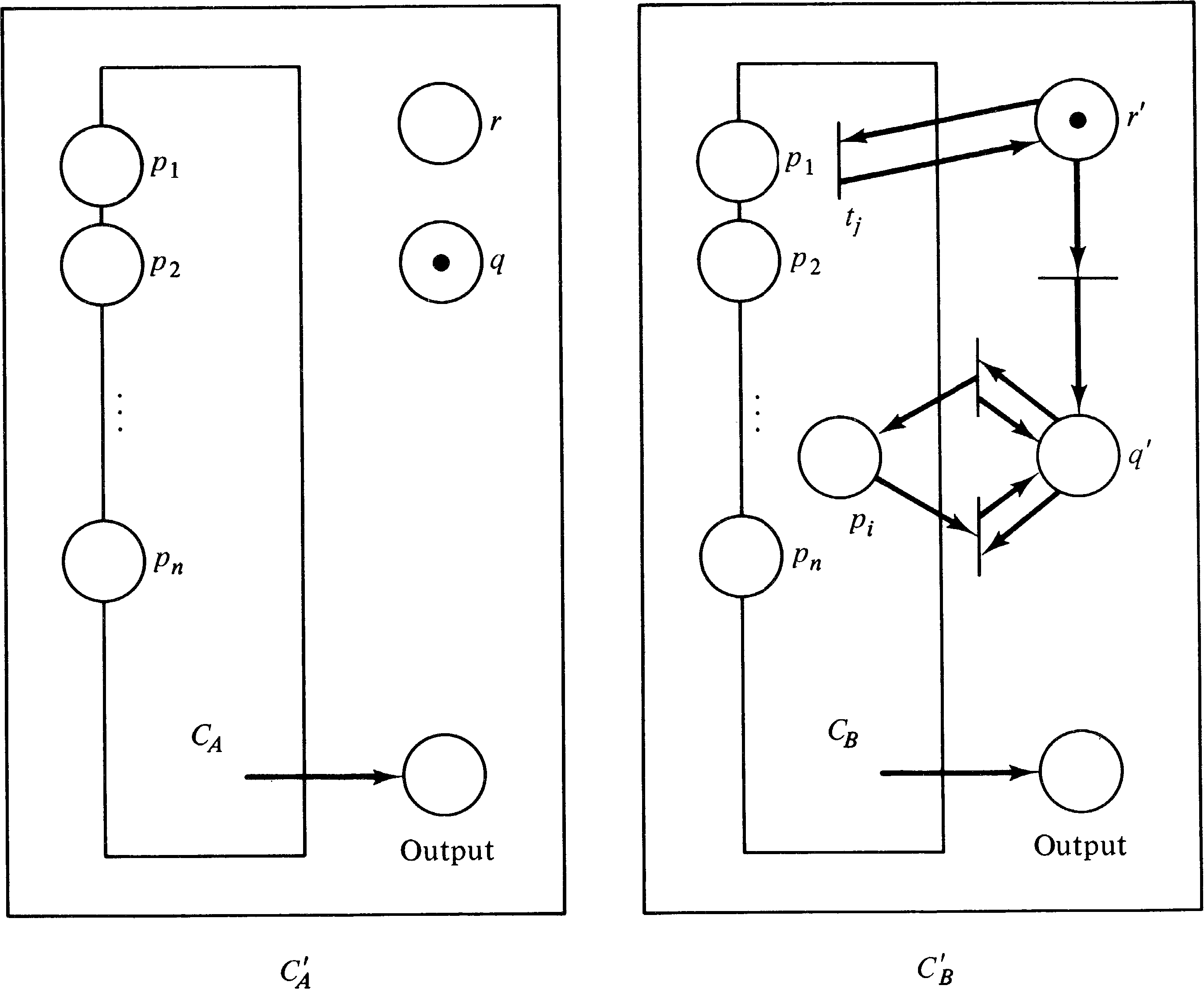Figure 5.13. The constructed Petri nets to test for polynomial graph inclusion.

The reachability sets of CA′ and CB′ are as follows. For CA′,

 p1 ... pn output r q internal places x1 ... xn y ≤ A(x1, …, xn) 0 1 Some arbitrary marking

For CB′,

 p1 ... pn output r q internal places x1 ⋯ xn y ≤ B(x1, …, xn) 1 0 Some arbitrary marking x1 ⋯ xn y ≤ B(x1, …, xn) 0 1 All arbitrary markings

Thus, if G ( A ) ⊆ G ( B ), then R ( CA′, μA′ ) ⊆ R ( CB′, μB′ ), and conversely, if R ( CA′, μA′ ) ⊆ R ( CB′, μB′ ), then G ( A ) ⊆ G ( B ) .

This concludes our demonstration of the following.

THEOREM 5.9
The polynomial graph inclusion problem is reducible to the subset problem for Petri net reachability sets.
This proof is from [Hack 1975a; Hack 1975c].

5.5.3. The Equality Problem

We now have only to show that the subset problem for Petri net reachability sets is reducible to the equality problem.

Assume that we have two Petri nets A and B and wish to determine if R ( A, μA ) ⊆ R ( B, μB ) (the subset problem). We now show that two Petri nets D and E can be defined such that R ( A, μA ) ⊆ R ( B, μB ) if and only if R ( D, μD ) = R ( E, μE ) . The basis for this construction is the fact that

 R(A, μA) ⊆ R(B, μB) if and only if R(B, μB) = R(A, μA) ∪ R(B, μB)

Both D and E are constructed from a common subnet, C. The net C encodes the reachability sets of both A and B in such a way as to produce their union. Figure 5.14 illustrates the basic construction. The n places p1, …, pn act as either the n places of net A or the n places of net B. Originally they are unmarked. Two new places rA and rB are added as “run” places for net A and net B, respectively. All transitions of net A are modified to include rA as both an input and an output; all transitions of net B are modified to include rB as both an input and an output.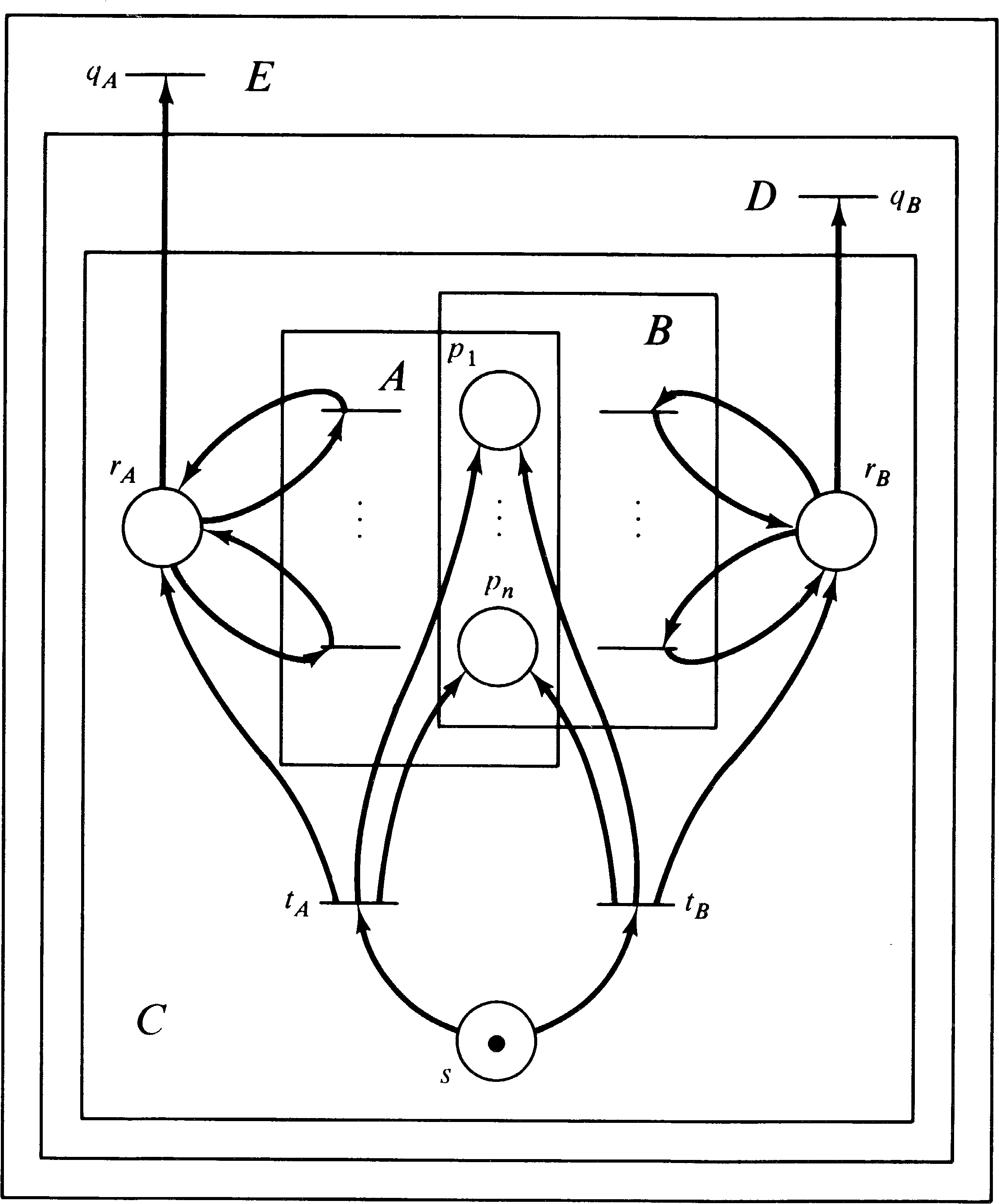Figure 5.14. The construction of Petri nets C, D, and E from A, and B. This construction is used to show that the subset problem is reducible to the equality problem for reachability sets.

Now, one more place, s, is added and two new transitions, tA and tB. The initial marking for this entire net (including A and B as subnets with shared places; places rA, rB, and s; and transitions tA and tB ) is one token in s and zero tokens elsewhere. Transition tA has place s as its input and as output produces the initial marking for net A plus a token in rA; transition tB has place s as its input and produces the initial marking for net B plus a token in rB. Thus, if tA fires, then the subnet A has its initial marking, and all of its transitions can fire as normal since there is a token in rA. However, subnet B is completely disabled, since there is no token in rB. If tB fires first, then the subnet B can operate, and A is disabled. The set of firing sequences for C is then any sequence of the form

 tA, < any sequence of firings from A>

or any sequence of the form

 tB, < any sequence of firings from B>

The net D is obtained from C by adding one new transition, qB. Transition qB has place rB as its input and no output. Notice that qB can fire only if transition tB was the first to fire; if transition tA fires first, then rB will be empty, and tB cannot fire.

The net E is constructed from D by adding a new transition, qA. Transition qA has place rA as its input and no output. Transition qA can fire only if tA was the first to fire: Notice that net E is constructed from D, not (directly) from C. So E has both transition qA and transition qB.

Now let us examine the reachability sets of the Petri nets C, D, and E. The reachability set of C is all markings of the form

 s rA rB p1, …, pn 1 0 0 0, …, 0(initial marking) 0 1 0 Any μ ∈ R(A, μA) (if tA fires) 0 0 1 Any μ ∈ R(B, μB) (if tB fires)

Petri net D adds one other class of markings to this set:

 s rA rB p1, …, pn 1 0 0 0, …, 0(initial marking) 0 1 0 Any μ ∈ R(A, μA) (if tA fires) 0 0 1 Any μ ∈ R(B, μB) (if tB fires) 0 0 0 Any μ ∈ R(B, μB) (if qB fires)

And Petri net E adds one more class to this:

 s rA rB p1, …, pn 1 0 0 0, …, 0(initial marking) 0 1 0 Any μ ∈ R(A, μA) (if tA fires) 0 0 1 Any μ ∈ R(B, μB) (if tB fires) 0 0 0 Any μ ∈ R(B, μB) (if qB fires) 0 0 0 Any μ ∈ R(A, μA) (if qA fires)

Now, if R ( A, μA ) ⊆ R ( B, μB ), the last class in R ( E, μE ) [markings of the form (0, 0, 0, μ ) with μ ∈ R ( A, μA ) ] is included in the last class of R ( D, μD ) [markings of the form (0, 0, 0, μ ) with μ ∈ R ( B, μB ) ]. Since all other markings are the same,

 R(D, μD) = R(E, μE) if R(A, μA) ⊆ R(B, μB)

Similarly, if R ( D, μD ) = R ( E, μE ), then we must have R ( A, μA ) ⊆ R ( B, μB ), since for each (0, 0, 0, μ ) with μ ∈ R ( A, μA ) in R ( E, μE ) there must exist an equal marking in R ( D, μD ) . But all markings with μ ( s, rA, rB ) = (0, 0, 0) are of the form (0, 0, 0, μ ) with μ ∈ R ( B, μB ), so R ( A, μA ) ⊆ R ( B, μB ) .

Thus, this construction shows the following.

THEOREM 5.10
The subset problem for Petri net reachability sets is reducible to the equality problem for Petri net reachability sets.
These three theorems then lead to the following.
THEOREM 5.11
The following problems are undecidable.

1. The polynomial graph inclusion problem

2. The subset problem for Petri net reachability sets

3. The equality problem for Petri net reachability sets
These theorems and their proofs are due to Hack [1975a; 1975c].

5.6. Complexity of the Reachability Problem

The undecidability of the subset and equality problems for Petri net reachability sets creates the possibility that the reachability problem itself is also undecidable. However, at the moment, the decidability (or undecidability) of the reachability problem is open. There is currently neither an algorithm to solve the reachability problem nor a proof that such an algorithm cannot exist.

In 1977, a “proof” of the decidability of the reachability problem was presented at the ACM Symposium on Theory of Computing [Sacerdote and Tenney 1977]. However, this “proof” has several serious flaws, and attempts to correct them, to produce a correct proof, have been unsuccessful. Still the prevailing feeling is that the reachability problem is decidable -- it is believed that an algorithm does exists and will be discovered in time.

Assuming that an algorithm to solve the reachability problem does exist, it is likely to be very complex. The obvious question is, If an algorithm to solve the reachability problem exists, how complex must it be? Some bounds on this complexity can be established without reference to any specific algorithm

Lipton  has shown that any algorithm to solve the reachability problem will require at least an exponential ( 2 cn ) amount of space for storage and an exponential amount of time. The exponent ( n ) is a measure of the size of the problem and in Lipton's case reflects the number of places and their interconnections to transitions.

Lipton proved that exponential space is necessary by showing that a Petri net can be constructed in which a place acts as a counter of the numbers 0, 1, …, 22n. Representing this in the reachability problem algorithm would require at least log2 (22n ) = 2n bits. Just as important is that his construction uses at most hn places (for some constant h ).

Lipton's proof hinges on the ability to create a net to count to 22n in only hn places. Part of the constraints is a need to test this place for zero. Petri nets, of course, have been designed so that there is no direct way to test for zero. However, a common technique used with Petri nets to allow zero testing is to use two places p and p′ such that μ ( p ) + μ ( p′ ) is a constant. If we know that μ ( p ) + μ ( p′ ) = k, then we can test for μ ( p ) being zero by testing if μ ( p′ ) has k tokens; if μ ( p′ ) has k tokens, then μ ( p ) has zero tokens and vice versa. A place can be tested for nonzero by using it in a self-loop. Note that to maintain this ability we must maintain the constant nature of μ ( p ) + μ ( p′ ) ; that is, the net must be conservative, at least with respect to these two places.

For small numbers k one can test if the marking of a place is k by having the place be an input to a transition k times (Figure 5.15). However, these arcs contribute to the size of the problem, and so we cannot do this in general. Lipton showed that if the constant sum of two places ( pk, pk′ ) is k and k is a product of two smaller integer factors k = k1k2 which are the constant sums of two other pairs of places ( p k1, p k1′ and p k2, p k2′ ) and we can test μ ( p k1 ) = 0 and μ ( p k2 ) = 0, then we can test if μ ( pk ) = 0 . This allowed Lipton to build subnets such as Figure 5.16. These nets are then used to control multiplication nets, similar to the nets used to weakly compute the polynomial graph (see Figure 5.10). The test-for-zero subnet allows the Petri net to compute the exact product (not a weak product which is merely bounded by the real product).Figure 5.15. Testing a bounded place for a marking of 0, 1 or 2. All transitions must maintain the sum of the markings of p and p′ at 2.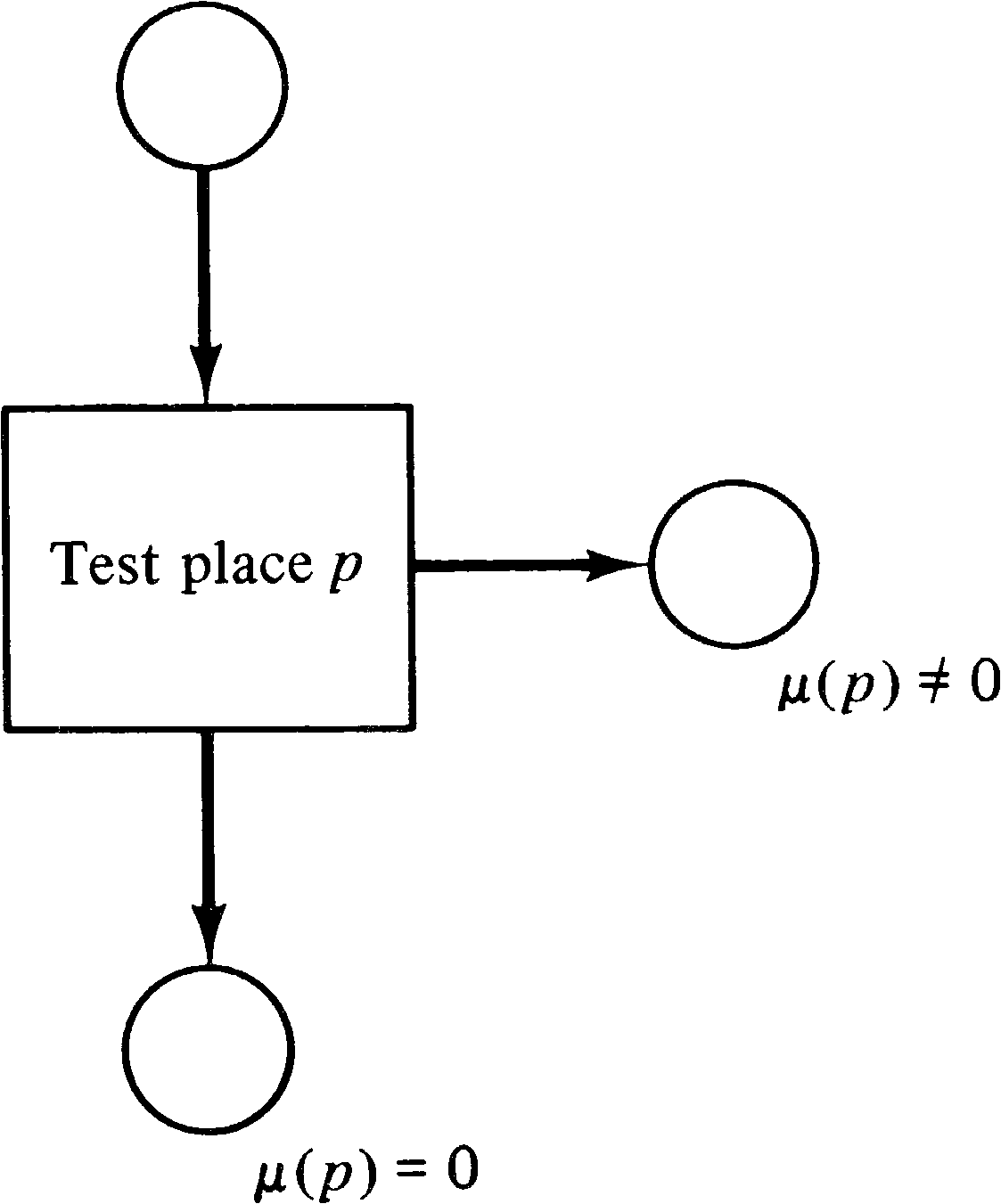Figure 5.16. The form of the Petri nets which Lipton uses to construct a larger net which can test a larger counter for zero.

These simple nets allow Lipton to build a net, for a given n, which can generate exactly 22n tokens in a place ( p ) with zero tokens in p′ and the ability to test μ ( p ) for zero. The number of places used is only a constant factor times n. The existence of a Petri net like this shows that the reachability problem requires exponential time and space and hence will be very expensive to solve.

The construction of a Petri net which can count up to 22n has a very important corollary, too. The Petri net which is constructed is bounded, since the number of tokens in any given place cannot exceed 22n. This means that any algorithm to determine boundedness of a Petri net must also require exponential time and space. Thus, even simple problems for Petri nets, while decidable, may require large amounts of time and space for solution.

It should be remembered that these are lower bounds on the worst-case behavior of an algorithm. It may be the case that many interesting problems can be decided for most Petri nets relatively efficiently. These complexity results show that even if an algorithm works very well most of the time there exists a Petri net which will take lots of time and space to analyze.

Although these are worst-case complexity results (which means the average case may be much better), they are also lower-bound results. We know that the reachability problem requires exponential space, at least. It may be that reachability is even worse than exponential. Rackoff  has developed an algorithm for determining boundedness in exponential time, so the boundedness problem is known to be of exponential complexity. However, the reachability problem is simply known to be at least exponentially complex (and may not even be decidable).

A recent result by Mayr  showed that the subset and equality problems for bounded Petri net reachability sets are of nonprimitive recursive complexity. These results indicate that some problems for Petri nets, while decidable, are computationally intractable.

Exercises

1. Show that the reachability problem for ordinary Petri nets is equivalent to the single-place zero-reachability problem for self-loop-free Petri nets.

2. For a Petri net C1 = (P1, T1, I1, O1 ), define a new Petri net C2 = (P2, T2, I2, O2 ) with

 P2 = P1 ∪ { pj′ | tj ∈ T1 } T2 = T1 I2 = I1 O2(tj) = O1(tj) ∪ { pj′ }

This introduces one extra place as an output of each transition.

1. What is the meaning of the number of tokens in each of these places? For a live Petri net, what is the bound on the marking of these places?

2. Suppose we add one extra transition with each pj′ as an input and no output. Show that the net is live if and only if this new transition is live.

Computability theory is an early part of the theory of computation and developed from the work of Turing, Kleene, Godel, and Church. Davis  and Minsky  offer good explanations of this work. Karp  shows how reducibility can be used for decidability and complexity results.

The reachability problem first arose in [Karp and Miller 1968]; it was reported as a research question in [Nash 1973]. Preliminary results were reported in [Van Leeuwen 1974; Hopcroft and Pansiot 1976], but these do not generalize.

Most of the results in this chapter are due to the work of Hack [1974a; 1974c; 1975a; 1975c]. Hack has been one of the major researchers on decision problems for Petri nets. Other work on decision properties includes [Araki and Kasami 1976; Araki and Kasami 1977; Mayr 1977]. Complexity results have been produced by Lipton , Rackoff , and Jones et al.  among others. Some related work not directly tied to Petri nets is [Cardoza 1975; Cardoza et al. 1976].

5.8. Topics for Further Study

1. A Petri net is reversible if for every transition tjT there exists tkT such that

 #(pi, I(tj)) = #(pi, O(tk)) #(pi, O(tj)) = #(pi, I(tk))

That is, for every transition there is another transition with inputs and outputs reversed. This allows any sequence of transitions to be “undone” by firing their complementary transitions in the opposite order. It has been stated [Hopcroft and Pansiot 1976] that the reachability and equivalence problems are decidable for reversible Petri nets. This theorem is based on work with commutative semigroups [Cardoza 1975]. Follow this statement up, showing the relationship between reversible Petri nets and commutative semigroups, and establish the decidability of reachability and equivalence for reversible Petri nets. Also consider the liveness problem, complexity issues, and the languages of reversible Petri nets to develop a theory of reversible Petri nets.

2. There would seem to be a very useful connection between Petri nets and Presburger arithmetic. Presburger arithmetic is a theory of arithmetic using addition and subtraction with integers. It has been shown that it is possible to determine the truth or falseness of all statements formed from first-order quantifiers, equality, the operations of addition and subtraction, and integers. The original proof was presented in [Presburger 1929] and has been used as the basis of theorem-proving programs [Davis 1957; Cooper 1971]. The connection of Presburger arithmetic to semilinear sets was mentioned in [Ginsburg 1966; Ginsburg and Spanier 1966], and the relationship of semilinear sets to Petri net reachability has been mentioned in [Van Leeuwen 1974; Crespi-Reghizzi and Mandrioli 1974; Landweber and Robertson 1975; Hopcroft and Pansiot 1976; Jaffe 1977]. I suspect that Presburger arithmetic can be used to solve analysis problems for Petri nets. Investigate the usefulness of Presburger arithmetic in the analysis of Petri nets.